• 各位好！在使用python2.7画图的时候，plt.show()总是无限弹出另存为窗口?如果不关闭图片，一直会无限弹...（做任何图都是），IDE:pycharm 有时候重启电脑再跑就不会弹出另存为窗口，但是一段时间后又会出现问题。迷惑。python pycharm
• 如何根据图表中没有的变量更改折线图的背景颜色？例如，如果我有以下数据帧： ...如果我做df.A的折线图，如何根据该时间点’B’列的值更改背景颜色？ 例如，如果在该日期B = 1，则该日期的背景为绿色
• 但是由于matplotlib中的显示模式是阻塞模式，在plt.show()之后程序就会暂停在那，打开一个窗口以后必须关掉才能继续执行，这样用matplotlib画动态图就像播放ppt，关闭一个窗口后弹出下一个。关于matplotlib包的任何...
• 文章目录matplotlib概述matplotlib基本功能matplotlib基本功能详解基本绘图绘图核心API线型、线宽和颜色设置坐标轴范围设置坐标刻度设置坐标轴图例特殊点备注图形对象（图形窗口）子图刻度定位器刻度网格线半对数...


文章目录
matplotlib概述matplotlib基本功能详解基本绘图绘图核心API线型、线宽和颜色设置坐标轴范围设置坐标刻度设置坐标轴图例特殊点备注
图形对象（图形窗口）子图刻度定位器刻度网格线半对数坐标散点图填充条形图（柱状图）饼图等高线图热成像图极坐标系3D图像绘制简单动画

附录matplotlib colors颜色字符串matplotlib point样式laTeX语法表示数学符号示例LaTeX语法集合cmap颜色映射表

matplotlib概述
matplotlib是python的一个绘图库。使用它可以很方便的绘制出版质量级别的图形。
matplotlib基本功能详解
基本绘图
绘图核心API
案例：绘制一条余弦曲线
import numpy as np
import matplotlib.pyplot as mp

# xarray: <序列> 水平坐标序列
# yarray: <序列> 垂直坐标序列
mp.plot(xarray, yarray)
#显示图表
mp.show()

绘制水平线与垂直线：
import numpy as np
import matplotlib.pyplot as mp

# vertical 绘制垂直线
mp.vlines(vval, ymin, ymax, ...)
# horizotal 绘制水平线
mp.hlines(xval, xmin, xmax, ...)
#显示图表
mp.show()

线型、线宽和颜色
案例：绘制一条正弦曲线

#	数字
#color: <关键字参数> 颜色
#	英文颜色单词 或 常见颜色英文单词首字母 或 #495434 或 (1,1,1) 或 (1,1,1,1)
#alpha: <关键字参数> 透明度
#	浮点数值
mp.plot(xarray, yarray, linestyle='', linewidth=1, color='', alpha=0.5)

设置坐标轴范围
案例：把坐标轴范围设置为 -π ~ π
#x_limt_min:	<float> x轴范围最小值
#x_limit_max:	<float> x轴范围最大值
mp.xlim(x_limt_min, x_limit_max)
#y_limt_min:	<float> y轴范围最小值
#y_limit_max:	<float> y轴范围最大值
mp.ylim(y_limt_min, y_limit_max)

设置坐标刻度
案例：把横坐标的刻度显示为：0, π/2, π, 3π/2, 2π
#x_val_list: 	x轴刻度值序列
#x_text_list:	x轴刻度标签文本序列 [可选]
mp.xticks(x_val_list , x_text_list )
#y_val_list: 	y轴刻度值序列
#y_text_list:	y轴刻度标签文本序列 [可选]
mp.yticks(y_val_list , y_text_list )

刻度文本的特殊语法 – LaTex排版语法字符串
r'$x^n+y^n=z^n$',   r'$\int\frac{1}{x} dx = \ln |x| + C$',     r'$-\frac{\pi}{2}$'


x

n

+

y

n

=

z

n

,

∫

1

x

d

x

=

ln

⁡

∣

x

∣

+

C

,

−

π

2

x^n+y^n=z^n, \int\frac{1}{x} dx = \ln |x| + C, -\frac{\pi}{2}

设置坐标轴
坐标轴名：left / right / bottom / top
# 获取当前坐标轴字典，{'left':左轴,'right':右轴,'bottom':下轴,'top':上轴 }
ax = mp.gca()
# 获取其中某个坐标轴
axis = ax.spines['坐标轴名']
# 设置坐标轴的位置。 该方法需要传入2个元素的元组作为参数
# type: <str> 移动坐标轴的参照类型  一般为'data' (以数据的值作为移动参照值)
# val:  参照值
axis.set_position((type, val))
# 设置坐标轴的颜色
# color: <str> 颜色值字符串
axis.set_color(color)

案例：设置坐标轴至中心。
#设置坐标轴
ax = mp.gca()
axis_b = ax.spines['bottom']
axis_b.set_position(('data', 0))
axis_l = ax.spines['left']
axis_l.set_position(('data', 0))
ax.spines['top'].set_color('none')
ax.spines['right'].set_color('none')

图例
显示两条曲线的图例，并测试loc属性。
# 再绘制曲线时定义曲线的label
# label: <关键字参数 str> 支持LaTex排版语法字符串
mp.plot(xarray, yarray ... label='', ...)
# 设置图例的位置
# loc: <关键字参数> 制定图例的显示位置 (若不设置loc，则显示默认位置)
#	 ===============   =============
#    Location String   Location Code
#    ===============   =============
#    'best'            0
#    'upper right'     1
#    'upper left'      2
#    'lower left'      3
#    'lower right'     4
#    'right'           5
#    'center left'     6
#    'center right'    7
#    'lower center'    8
#    'upper center'    9
#    'center'          10
#    ===============   =============
mp.legend(loc='')

特殊点
案例：绘制当x=3π/4时两条曲线上的特殊点。
# xarray: <序列> 所有需要标注点的水平坐标组成的序列
# yarray: <序列> 所有需要标注点的垂直坐标组成的序列
mp.scatter(xarray, yarray,
marker='', 		#点型 ~ matplotlib.markers
s='', 			#大小
edgecolor='', 	#边缘色
facecolor='',	#填充色
zorder=3			#绘制图层编号 （编号越大，图层越靠上）
)

marker点型可参照：help(matplotlib.markers)
也可参照附录： matplotlib point样式
备注
案例：为在某条曲线上的点添加备注，指明函数方程与值。
# 在图表中为某个点添加备注。包含备注文本，备注箭头等图像的设置。
mp.annotate(
r'$\frac{\pi}{2}$',			#备注中显示的文本内容
xycoords='data',			#备注目标点所使用的坐标系（data表示数据坐标系）
xy=(x, y),	 				#备注目标点的坐标
textcoords='offset points',	#备注文本所使用的坐标系（offset points表示参照点的偏移坐标系）
xytext=(x, y),				#备注文本的坐标
fontsize=14,				#备注文本的字体大小
arrowprops=dict()			#使用字典定义文本指向目标点的箭头样式
)

arrowprops参数使用字典定义指向目标点的箭头样式
#arrowprops字典参数的常用key
arrowprops=dict(
arrowstyle='',		#定义箭头样式
connectionstyle=''	#定义连接线的样式
)

箭头样式（arrowstyle）字符串如下
============   =============================================
Name           Attrs
============   =============================================
'-'          None
'-['         widthB=1.0,lengthB=0.2,angleB=None
'|-|'        widthA=1.0,widthB=1.0
'wedge'      tail_width=0.3,shrink_factor=0.5
============   =============================================

连接线样式（connectionstyle）字符串如下
============   =============================================
Name           Attrs
============   =============================================
'angle3' 		angleA=90,angleB=0
'bar' 		armA=0.0,armB=0.0,fraction=0.3,angle=None
============   =============================================

图形对象（图形窗口）
案例：绘制两个窗口，一起显示。
# 手动构建 matplotlib 窗口
mp.figure(
'',					#窗口标题栏文本
figsize=(4, 3),		#窗口大小 <元组>
dpi=120,			#像素密度
facecolor=''		#图表背景色
)
mp.show()

mp.figure方法不仅可以构建一个新窗口，如果已经构建过title='xxx’的窗口，又使用figure方法构建了title=‘xxx’ 的窗口的话，mp将不会创建新的窗口，而是把title='xxx’的窗口置为当前操作窗口。
设置当前窗口的参数
案例：测试窗口相关参数
# 设置图表标题 显示在图表上方
mp.title(title, fontsize=12)
# 设置水平轴的文本
mp.xlabel(x_label_str, fontsize=12)
# 设置垂直轴的文本
mp.ylabel(y_label_str, fontsize=12)
# 设置刻度参数   labelsize设置刻度字体大小
mp.tick_params(..., labelsize=8, ...)
# 设置图表网格线  linestyle设置网格线的样式
#	-  or solid 粗线
#   -- or dashed 虚线
#   -. or dashdot 点虚线
#   :  or dotted 点线
mp.grid(linestyle='')
# 设置紧凑布局，把图表相关参数都显示在窗口中
mp.tight_layout()

子图
矩阵式布局
绘制矩阵式子图布局相关API：
mp.figure('Subplot Layout', facecolor='lightgray')
# 拆分矩阵
# rows:	行数
# cols:	列数
# num:	编号
mp.subplot(rows, cols, num)
#	1 2 3
#	4 5 6
#	7 8 9
mp.subplot(3, 3, 5)		#操作3*3的矩阵中编号为5的子图
mp.subplot(335)			#简写

案例：绘制9宫格矩阵式子图，每个子图中写一个数字。
mp.figure('Subplot Layout', facecolor='lightgray')

for i in range(9):
mp.subplot(3, 3, i+1)
mp.text(
0.5, 0.5, i+1,
ha='center',
va='center',
size=36,
alpha=0.5,
withdash=False
)
mp.xticks([])
mp.yticks([])

mp.tight_layout()
mp.show()

网格式布局
网格式布局支持单元格的合并。
绘制网格式子图布局相关API：
import matplotlib.gridspec as mg
mp.figure('Grid Layout', facecolor='lightgray')
# 调用GridSpec方法拆分网格式布局
# rows:	行数
# cols:	列数
# gs = mg.GridSpec(rows, cols)	拆分成3行3列
gs = mg.GridSpec(3, 3)
# 合并0行与0、1列为一个子图表
mp.subplot(gs[0, :2])
mp.text(0.5, 0.5, '1', ha='center', va='center', size=36)
mp.show()

案例：绘制一个自定义网格布局。
import matplotlib.gridspec as mg
mp.figure('GridLayout', facecolor='lightgray')
gridsubs = mp.GridSpec(3, 3)
# 合并0行、0/1列为一个子图
mp.subplot(gridsubs[0, :2])
mp.text(0.5, 0.5, 1, ha='center', va='center', size=36)
mp.tight_layout()
mp.xticks([])
mp.yticks([])

自由式布局
自由式布局相关API：
mp.figure('Flow Layout', facecolor='lightgray')
# 设置图标的位置，给出左下角点坐标与宽高即可
# left_bottom_x: 坐下角点x坐标
# left_bottom_x: 坐下角点y坐标
# width:		 宽度
# height:		 高度
# mp.axes([left_bottom_x, left_bottom_y, width, height])
mp.axes([0.03, 0.03, 0.94, 0.94])
mp.text(0.5, 0.5, '1', ha='center', va='center', size=36)
mp.show()

案例：测试自由式布局，定位子图。
mp.figure('FlowLayout', facecolor='lightgray')

mp.axes([0.1, 0.2, 0.5, 0.3])
mp.text(0.5, 0.5, 1, ha='center', va='center', size=36)
mp.show()

刻度定位器
刻度定位器相关API：
# 获取当前坐标轴
ax = mp.gca()
# 设置水平坐标轴的主刻度定位器
ax.xaxis.set_major_locator(mp.NullLocator())
# 设置水平坐标轴的次刻度定位器为多点定位器，间隔0.1
ax.xaxis.set_minor_locator(mp.MultipleLocator(0.1))

案例：绘制一个数轴。
mp.figure('Locators', facecolor='lightgray')
# 获取当前坐标轴
ax = mp.gca()
# 隐藏除底轴以外的所有坐标轴
ax.spines['left'].set_color('none')
ax.spines['top'].set_color('none')
ax.spines['right'].set_color('none')
# 将底坐标轴调整到子图中心位置
ax.spines['bottom'].set_position(('data', 0))
# 设置水平坐标轴的主刻度定位器
ax.xaxis.set_major_locator(mp.NullLocator())
# 设置水平坐标轴的次刻度定位器为多点定位器，间隔0.1
ax.xaxis.set_minor_locator(mp.MultipleLocator(0.1))
# 标记所用刻度定位器类名
mp.text(5, 0.3, 'NullLocator()', ha='center', size=12)

案例：使用for循环测试刻度器样式：
locators = ['mp.NullLocator()', 'mp.MaxNLocator(nbins=4)']

for i, locator in enumerate(locators):
mp.subplot(len(locators), 1, i+1)
mp.xlim(0, 10)
mp.ylim(-1, 1)
mp.yticks([])
# 获取当前坐标轴
ax = mp.gca()
# 隐藏除底轴以外的所有坐标轴
ax.spines['left'].set_color('none')
ax.spines['top'].set_color('none')
ax.spines['right'].set_color('none')
# 将底坐标轴调整到子图中心位置
ax.spines['bottom'].set_position(('data', 0))
# 设置水平坐标轴的主刻度定位器
ax.xaxis.set_major_locator(eval( ))
# 设置水平坐标轴的次刻度定位器为多点定位器，间隔0.1
ax.xaxis.set_minor_locator(mp.MultipleLocator(0.1))
mp.plot(np.arange(11), np.zeros(11), c='none')
# 标记所用刻度定位器类名
mp.text(5, 0.3, locator, ha='center', size=12)

常用刻度器如下
# 空定位器：不绘制刻度
mp.NullLocator()
# 最大值定位器：
# 最多绘制nbins+1个刻度
mp.MaxNLocator(nbins=3)
# 定点定位器：根据locs参数中的位置绘制刻度
mp.FixedLocator(locs=[0, 2.5, 5, 7.5, 10])
# 自动定位器：由系统自动选择刻度的绘制位置
mp.AutoLocator()
# 索引定位器：由offset确定起始刻度，由base确定相邻刻度的间隔
mp.IndexLocator(offset=0.5, base=1.5)
# 多点定位器：从0开始，按照参数指定的间隔(缺省1)绘制刻度
mp.MultipleLocator()
# 线性定位器：等分numticks-1份，绘制numticks个刻度
mp.LinearLocator(numticks=21)
# 对数定位器：以base为底，绘制刻度
mp.LogLocator(base=2)

刻度网格线
绘制刻度网格线的相关API：
ax = mp.gca()
#绘制刻度网格线
ax.grid(
which='',		# 'major'/'minor' <-> '主刻度'/'次刻度'
axis='',		# 'x'/'y'/'both' <-> 绘制x或y轴
linewidth=1, 	# 线宽
linestyle='', 	# 线型
color='',		# 颜色
alpha=0.5		# 透明度
)

案例：绘制曲线 [1, 10, 100, 1000, 100, 10, 1]，然后设置刻度网格线，测试刻度网格线的参数。
y = np.array([1, 10, 100, 1000, 100, 10, 1])
mp.figure('Normal & Log', facecolor='lightgray')
mp.subplot(211)
mp.title('Normal', fontsize=20)
mp.ylabel('y', fontsize=14)
ax = mp.gca()
ax.xaxis.set_major_locator(mp.MultipleLocator(1.0))
ax.xaxis.set_minor_locator(mp.MultipleLocator(0.1))
ax.yaxis.set_major_locator(mp.MultipleLocator(250))
ax.yaxis.set_minor_locator(mp.MultipleLocator(50))
mp.tick_params(labelsize=10)
ax.grid(which='major', axis='both', linewidth=0.75,
linestyle='-', color='orange')
ax.grid(which='minor', axis='both', linewidth=0.25,
linestyle='-', color='orange')
mp.plot(y, 'o-', c='dodgerblue', label='plot')
mp.legend()

半对数坐标
y轴将以指数方式递增。 基于半对数坐标绘制第二个子图，表示曲线：[1, 10, 100, 1000, 100, 10, 1]。
mp.figure('Grid', facecolor='lightgray')
y = [1, 10, 100, 1000, 100, 10, 1]
mp.semilogy(y)
mp.show()

散点图
可以通过每个点的坐标、颜色、大小和形状表示不同的特征值。
身高体重性别年龄段种族18080男中年亚洲16050女青少美洲
绘制散点图的相关API：
mp.scatter(
x, 					# x轴坐标数组
y,					# y轴坐标数组
marker='', 			# 点型
s=10,				# 大小
color='',			# 颜色
edgecolor='', 		# 边缘颜色
facecolor='',		# 填充色
zorder=''			# 图层序号
)

numpy.random提供了normal函数用于产生符合 正态分布 的随机数
n = 100
# 172:	期望值
# 10:	标准差
# n:	数字生成数量
x = np.random.normal(172, 20, n)
y = np.random.normal(60, 10, n)

案例：绘制平面散点图。
mp.figure('scatter', facecolor='lightgray')
mp.title('scatter')
mp.scatter(x, y)
mp.show()

设置点的颜色
mp.scatter(x, y, c='red')			#直接设置颜色
d = (x-172)**2 + (y-60)**2
mp.scatter(x, y, c=d, cmap='jet')	#以c作为参数，取cmap颜色映射表中的颜色值

cmap颜色映射表参照附件：cmap颜色映射表
填充
以某种颜色自动填充两条曲线的闭合区域。
mp.fill_between(
x,				# x轴的水平坐标
sin_x,			# 下边界曲线上点的垂直坐标
cos_x,			# 上边界曲线上点的垂直坐标
sin_x<cos_x, 	# 填充条件，为True时填充
color='', 		# 填充颜色
alpha=0.2		# 透明度
)

案例：绘制两条曲线： sin_x = sin(x) cos_x = cos(x / 2) / 2 [0-8π]
n = 1000
x = np.linspace(0, 8 * np.pi, n)
sin_y = np.sin(x)
cos_y = np.cos(x / 2) / 2
mp.figure('Fill', facecolor='lightgray')
mp.title('Fill', fontsize=20)
mp.xlabel('x', fontsize=14)
mp.ylabel('y', fontsize=14)
mp.tick_params(labelsize=10)
mp.grid(linestyle=':')
mp.plot(x, sin_y, c='dodgerblue',
label=r'$y=sin(x)$')
mp.plot(x, cos_y, c='orangered',
label=r'$y=\frac{1}{2}cos(\frac{x}{2})$')
mp.fill_between(x, cos_y, sin_y, cos_y < sin_y,
color='dodgerblue', alpha=0.5)
mp.fill_between(x, cos_y, sin_y, cos_y > sin_y,
color='orangered', alpha=0.5)
mp.legend()
mp.show()

条形图（柱状图）
绘制柱状图的相关API：
mp.figure('Bar', facecolor='lightgray')
mp.bar(
x,				# 水平坐标数组
y,				# 柱状图高度数组
width,			# 柱子的宽度
color='', 		# 填充颜色
label='',		#
alpha=0.2		#
)

案例：先以柱状图绘制苹果12个月的销量，然后再绘制橘子的销量。
apples = np.array([30, 25, 22, 36, 21, 29, 20, 24, 33, 19, 27, 15])
oranges = np.array([24, 33, 19, 27, 35, 20, 15, 27, 20, 32, 20, 22])
mp.figure('Bar'  , facecolor='lightgray')
mp.title('Bar', font size=20)
mp.xlabel('Month', fontsize=14)
mp.ylabel('Price', fontsize=14)
mp.tick_params(labelsize=10)
mp.grid(axis='y', linestyle=':')
mp.ylim((0, 40))
x = np.arange(len(apples))
mp.bar(x-0.2, apples, 0.4, color='dodgerblue',label='Apple')
mp.bar(x + 0.2, oranges, 0.4, color='orangered',label='Orange', alpha=0.75)
mp.xticks(x, [
'Jan', 'Feb', 'Mar', 'Apr', 'May', 'Jun',
'Jul', 'Aug', 'Sep', 'Oct', 'Nov', 'Dec'])
mp.legend()
mp.show()

饼图
绘制饼状图的基本API：
mp.pie(
values, 		# 值列表
spaces, 		# 扇形之间的间距列表
labels, 		# 标签列表
colors, 		# 颜色列表
'%d%%',			# 标签所占比例格式
startangle=90	# 逆时针绘制饼状图时的起始角度
)

案例：绘制饼状图显示5门语言的流行程度：
mp.figure('pie', facecolor='lightgray')
#整理数据
values = [26, 17, 21, 29, 11]
spaces = [0.05, 0.01, 0.01, 0.01, 0.01]
labels = ['Python', 'JavaScript',
'C++', 'Java', 'PHP']
colors = ['dodgerblue', 'orangered',
'limegreen', 'violet', 'gold']
mp.figure('Pie', facecolor='lightgray')
mp.title('Pie', fontsize=20)
# 等轴比例
mp.axis('equal')
mp.pie(
values, 		# 值列表
spaces, 		# 扇形之间的间距列表
labels, 		# 标签列表
colors, 		# 颜色列表
'%d%%',			# 标签所占比例格式
startanle=90	# 逆时针绘制饼状图时的起始角度
)

等高线图
组成等高线需要网格点坐标矩阵，也需要每个点的高度。所以等高线属于3D数学模型范畴。
绘制等高线的相关API：
mp.contourf(x, y, z, 8, cmap='jet')
cntr = mp.contour(
x, 					# 网格坐标矩阵的x坐标 （2维数组）
y, 					# 网格坐标矩阵的y坐标 （2维数组）
z, 					# 网格坐标矩阵的z坐标 （2维数组）
8, 					# 把等高线绘制成8部分
colors='black',		# 等高线的颜色
linewidths=0.5		# 线宽
)

案例：生成网格坐标矩阵，并且绘制等高线：
n = 1000
# 生成网格化坐标矩阵
x, y = np.meshgrid(np.linspace(-3, 3, n),
np.linspace(-3, 3, n))
# 根据每个网格点坐标，通过某个公式计算z高度坐标
z = (1 - x/2 + x**5 + y**3) * np.exp(-x**2 - y**2)
mp.figure('Contour', facecolor='lightgray')
mp.title('Contour', fontsize=20)
mp.xlabel('x', fontsize=14)
mp.ylabel('y', fontsize=14)
mp.tick_params(labelsize=10)
mp.grid(linestyle=':')
# 绘制等高线图
mp.contourf(x, y, z, 8, cmap='jet')
cntr = mp.contour(x, y, z, 8, colors='black',
linewidths=0.5)
# 为等高线图添加高度标签
mp.clabel(cntr, inline_spacing=1, fmt='%.1f',
fontsize=10)
mp.show()

热成像图
用图形的方式显示矩阵及矩阵中值的大小 1 2 3 4 5 6 7 8 9
绘制热成像图的相关API：
# 把矩阵z图形化，使用cmap表示矩阵中每个元素值的大小
# origin: 坐标轴方向
#    upper: 缺省值，原点在左上角
#    lower: 原点在左下角
mp.imshow(z, cmap='jet', origin='low')

使用颜色条显示热度值：
mp.colorbar()

极坐标系
与笛卡尔坐标系不同，某些情况下极坐标系适合显示与角度有关的图像。例如雷达等。极坐标系可以描述极径ρ与极角θ的线性关系。
mp.figure("Polar", facecolor='lightgray')
mp.gca(projection='polar')
mp.title('Porlar', fontsize=20)
mp.xlabel(r'$\theta$', fontsize=14)
mp.ylabel(r'$\rho$', fontsize=14)
mp.tick_params(labelsize=10)
mp.grid(linestyle=':')
mp.show()

在极坐标系中绘制曲线：
#准备数据
t = np.linspace(0, 4*np.pi, 1000)
r = 0.8 * t
mp.plot(t, r)
mp.show()

案例，在极坐标系中绘制正弦函数。 y=3 sin(6x)
x = np.linspace(0, 6*np.pi, 1000)
y = 3*np.sin(6*x)
mp.plot(x, y)

3D图像绘制
matplotlib支持绘制三维曲面。若希望绘制三维曲面，需要使用axes3d提供的3d坐标系。
from mpl_toolkits.mplot3d import axes3d
ax3d = mp.gca(projection='3d')   # class axes3d

matplotlib支持绘制三维点阵、三维曲面、三维线框图：
ax3d.scatter(..)		# 绘制三维点阵
ax3d.plot_surface(..)	# 绘制三维曲面
ax3d.plot_wireframe(..)	# 绘制三维线框图

3d散点图的绘制相关API：
ax3d.scatter(
x, 				# x轴坐标数组
y,				# y轴坐标数组
marker='', 		# 点型
s=10,			# 大小
zorder='',		# 图层序号
color='',		# 颜色
edgecolor='', 	# 边缘颜色
facecolor='',	# 填充色
c=v,			# 颜色值 根据cmap映射应用相应颜色
cmap=''			#
)

案例：随机生成3组坐标，程标准正态分布规则，并且绘制它们。
n = 1000
x = np.random.normal(0, 1, n)
y = np.random.normal(0, 1, n)
z = np.random.normal(0, 1, n)
d = np.sqrt(x ** 2 + y ** 2 + z ** 2)
mp.figure('3D Scatter')
ax = mp.gca(projection='3d')  # 创建三维坐标系
mp.title('3D Scatter', fontsize=20)
ax.set_xlabel('x', fontsize=14)
ax.set_ylabel('y', fontsize=14)
ax.set_zlabel('z', fontsize=14)
mp.tick_params(labelsize=10)
ax.scatter(x, y, z, s=60, c=d, cmap='jet_r', alpha=0.5)
mp.show()

3d平面图的绘制相关API：
ax3d.plot_surface(
x, 					# 网格坐标矩阵的x坐标 （2维数组）
y, 					# 网格坐标矩阵的y坐标 （2维数组）
z, 					# 网格坐标矩阵的z坐标 （2维数组）
rstride=30,			# 行跨距
cstride=30, 		# 列跨距
cmap='jet'			# 颜色映射
)

案例：绘制3d平面图
n = 1000
# 生成网格化坐标矩阵
x, y = np.meshgrid(np.linspace(-3, 3, n),
np.linspace(-3, 3, n))
# 根据每个网格点坐标，通过某个公式计算z高度坐标
z = (1 - x/2 + x**5 + y**3) * np.exp(-x**2 - y**2)
mp.figure('3D', facecolor='lightgray')

ax3d = mp.gca(projection='3d')
mp.title('3D', fontsize=20)
ax3d.set_xlabel('x', fontsize=14)
ax3d.set_ylabel('y', fontsize=14)
ax3d.set_zlabel('z', fontsize=14)
mp.tick_params(labelsize=10)
# 绘制3D平面图
# rstride: 行跨距
# cstride: 列跨距
ax3d.plot_surface(x,y,z,rstride=30,cstride=30, cmap='jet')

案例：3d线框图的绘制
# 绘制3D平面图
# rstride: 行跨距
# cstride: 列跨距
ax3d.plot_wireframe(x,y,z,rstride=30,cstride=30,
linewidth=1, color='dodgerblue')

简单动画
动画即是在一段时间内快速连续的重新绘制图像的过程。
matplotlib提供了方法用于处理简单动画的绘制。定义update函数用于即时更新图像。
import matplotlib.animation as ma
#定义更新函数行为
def update(number):
pass
# 每隔10毫秒执行一次update更新函数，作用于mp.gcf()当前窗口对象
# mp.gcf()：	获取当前窗口
# update：	更新函数
# interval：	间隔时间（单位：毫秒）
anim = ma.FuncAnimation(mp.gcf(), update, interval=10)
mp.show()

案例：随机生成各种颜色的100个气泡。让他们不断的增大。
#自定义一种可以存放在ndarray里的类型，用于保存一个球
ball_type = np.dtype([
('position', float, 2),  # 位置(水平和垂直坐标)
('size', float, 1),      # 大小
('growth', float, 1),    # 生长速度
('color', float, 4)])    # 颜色(红、绿、蓝和透明度)

#随机生成100个点对象
n = 100
balls = np.zeros(100, dtype=ball_type)
balls['position']=np.random.uniform(0, 1, (n, 2))
balls['size']=np.random.uniform(40, 70, n)
balls['growth']=np.random.uniform(10, 20, n)
balls['color']=np.random.uniform(0, 1, (n, 4))

mp.figure("Animation", facecolor='lightgray')
mp.title("Animation", fontsize=14)
mp.xticks
mp.yticks(())

sc = mp.scatter(
balls['position'][:, 0],
balls['position'][:, 1],
balls['size'],
color=balls['color'], alpha=0.5)

#定义更新函数行为
def update(number):
balls['size'] += balls['growth']
#每次让一个气泡破裂，随机生成一个新的
boom_ind = number % n
balls[boom_ind]['size']=np.random.uniform(40, 70, 1)
balls[boom_ind]['position']=np.random.uniform(0, 1, (1, 2))
# 重新设置属性
sc.set_sizes(balls['size'])
sc.set_offsets(balls['position'])

# 每隔30毫秒执行一次update更新函数，作用于mp.gcf()当前窗口对象
# mp.gcf()：	获取当前窗口
# update：		更新函数
# interval：	间隔时间（单位：毫秒）
anim = ma.FuncAnimation(mp.gcf(), update, interval=30)
mp.show()

使用生成器函数提供数据，实现动画绘制
在很多情况下，绘制动画的参数是动态获取的，matplotlib支持定义generator生成器函数，用于生成数据，把生成的数据交给update函数更新图像：
import matplotlib.animation as ma
#定义更新函数行为
def update(data):
t, v = data
...
pass

def generator():
yield t, v

# 每隔10毫秒将会先调用生成器，获取生成器返回的数据，
# 把生成器返回的数据交给并且调用update函数，执行更新图像函数
anim = ma.FuncAnimation(mp.gcf(), update, generator,interval=10)

案例：绘制信号曲线：y=sin(2 * π * t) * exp(sin(0.2 * π * t))，数据通过生成器函数生成，在update函数中绘制曲线。
mp.figure("Signal", facecolor='lightgray')
mp.title("Signal", fontsize=14)
mp.xlim(0, 10)
mp.ylim(-3, 3)
mp.grid(linestyle='--', color='lightgray', alpha=0.5)
pl = mp.plot([], [], color='dodgerblue', label='Signal')
pl.set_data([],[])

x = 0

def update(data):
t, v = data
x, y = pl.get_data()
x.append(t)
y.append(v)
#重新设置数据源
pl.set_data(x, y)
#移动坐标轴
if(x[-1]>10):
mp.xlim(x[-1]-10, x[-1])

def y_generator():
global x
y = np.sin(2 * np.pi * x) * np.exp(np.sin(0.2 * np.pi * x))
yield (x, y)
x += 0.05

anim = ma.FuncAnimation(mp.gcf(), update, y_generator, interval=20)
mp.tight_layout()
mp.show()

附录
matplotlib colors颜色字符串
matplotlib point样式
laTeX语法表示数学符号示例
LaTeX语法集合
cmap颜色映射表
[外链图片转存失败,源站可能有防盗链机制,建议将图片保存下来直接上传(img-uhmptVzj-1595262313129)(C:/Users/Administrator/Desktop/%E6%95%B0%E6%8D%AE%E5%88%86%E6%9E%90%E7%AC%94%E8%AE%B0/matplotlib_images/matplotlib_cmap.png)]
展开全文• 是jupyter notebook里的命令, 意思是将那些用matplotlib绘制的图显示在页面里而不是弹出一个窗口，终端输入jupyter notebook, 然后新建一个ipynb，然后就可以看到图片是处于"inline"状态了 import ...
目录
常用绘图命令
5.4.1 2D图表
5.3.1 3D图表

常用绘图命令
%matplotlib inline
是jupyter notebook里的命令, 意思是将那些用matplotlib绘制的图显示在页面里而不是弹出一个窗口，终端输入jupyter notebook, 然后新建一个ipynb，然后就可以看到图片是处于"inline"状态了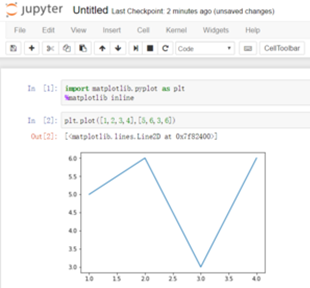import matplotlib.pyplot as plt import numpy as np import pandas as pd from pylab import mpl   fig=plt.figure() ax1=fig.add_subplot(2,2,1) ax2=fig.add_subplot(2,2,2) ax3=fig.add_subplot(2,2,3)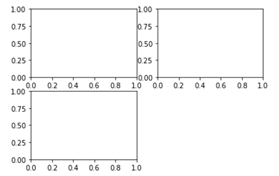from numpy.random import randn plt.plot(randn(50).cumsum(),'k--')   #cumsum()累计求和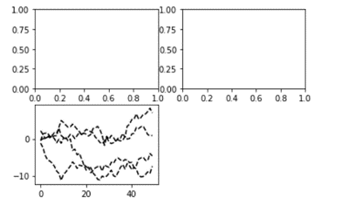ax1.hist(randn(100),bins=20,color='b',alpha=0.3) (array([  1.,   1.,   0.,   1.,   6.,   5.,   8.,  12.,  12.,   9.,   8.,          12.,   5.,   3.,   7.,   2.,   3.,   2.,   1.,   2.]),  array([-2.76369236, -2.48128569, -2.19887903, -1.91647236, -1.63406569,         -1.35165903, -1.06925236, -0.78684569, -0.50443902, -0.22203236,          0.06037431,  0.34278098,  0.62518765,  0.90759431,  1.19000098,          1.47240765,  1.75481431,  2.03722098,  2.31962765,  2.60203432,          2.88444098]),  <a list of 20 Patch objects>) fig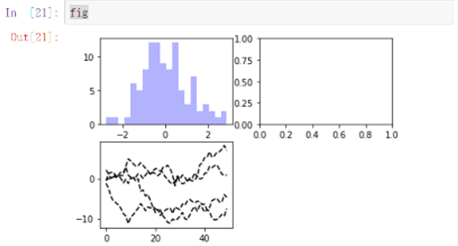ax2.scatter(np.arange(30),np.arange(30)+10*randn(30)) fig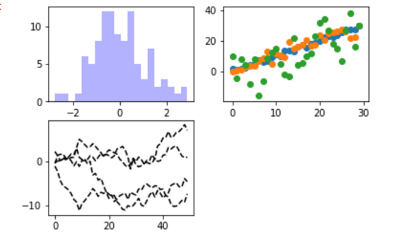fig2,axes=plt.subplots(2,3) axes array([[<matplotlib.axes._subplots.AxesSubplot object at 0x0000002EB19133C8>,         <matplotlib.axes._subplots.AxesSubplot object at 0x0000002EB1964F60>,         <matplotlib.axes._subplots.AxesSubplot object at 0x0000002EB199FF60>],        [<matplotlib.axes._subplots.AxesSubplot object at 0x0000002EB19D3EF0>,         <matplotlib.axes._subplots.AxesSubplot object at 0x0000002EB1A1A048>,         <matplotlib.axes._subplots.AxesSubplot object at 0x0000002EB19B1518>]], dtype=object) fig2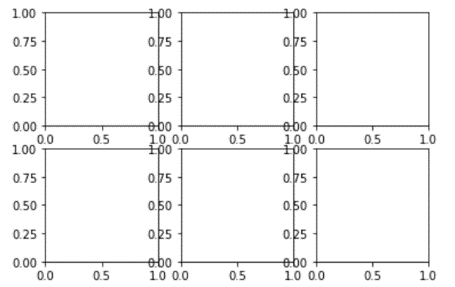nrows  subplot行数  ncols  subplot列数  sharex  所有subplot使用相同x刻度  sharey  相同y刻度  subplot_kw  创建各个subplot的关键字字典  **fig_kw  创建figure时的其他关键字，如plt.subplots(2,2,figsize(8,6))  调整subplot周围间距 subplots_adjust(left=none,bottom=None,right=None,top=None,wspace=None,hspace=None)   fig3, axes=plt.subplots(2,3,sharex=True,sharey=True) for i in range(2):     for j in range(2):         axes[i,j].hist(randn(500),bins=50,alpha=0.5) fig3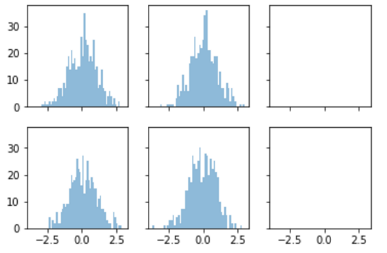fig3.subplots_adjust(wspace=0)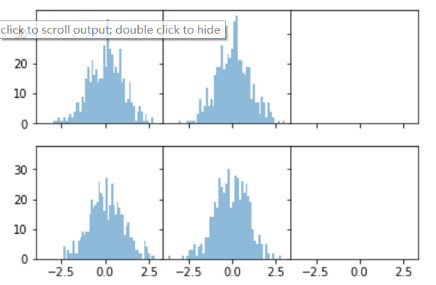fig3.subplots_adjust(hspace=0)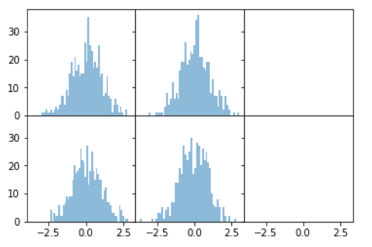需要掌握的是：
散点图，折线图，条形图，直方图，饼状图，箱形图的绘制。绘图的三大系统：pyplot，pylab(不推荐)，面向对象坐标轴的调整，添加文字注释，区域填充，及特殊图形patches的使用金融的同学注意的是：可以直接调用Yahoo财经数据绘图（真。。。）

5.4.1 2D图表 Matplotlib中最基础的模块是pyplot。先从最简单的点图和线图开始，比如我们有一组数据，还有一个拟合模型，通过下面的代码图来可视化： import numpy as np import matplotlib as mpl import matplotlib.pyplot as plt # 通过rcParams设置全局横纵轴字体大小 mpl.rcParams['xtick.labelsize'] = 24 mpl.rcParams['ytick.labelsize'] = 24   np.random.seed(42) ‘’’# seed( ) 用于指定随机数生成时所用算法开始的整数值，如果使用相同的seed( )值，则每次生成的随即数都相同，如果不设置这个值，则系统根据时间来自己选择这个值，此时每次生成的随机数因时间差异而不同。   # x轴的采样点 x = np.linspace(0, 5, 100) # 通过下面曲线加上噪声生成数据，所以拟合模型就用y了…… y = 2*np.sin(x) + 0.3*x**2 y_data = y + np.random.normal(scale=0.3, size=100)   # figure()指定图表名称 plt.figure('data') # '.'标明画散点图，每个散点的形状是个圆 plt.plot(x, y_data, '.') # 画模型的图，plot函数默认画连线图 plt.figure('model') plt.plot(x, y)   # 两个图画一起 plt.figure('data & model') # 通过'k'指定线的颜色，lw指定线的宽度 # 第三个参数除了颜色也可以指定线形，比如'r--'表示红色虚线 # 更多属性可以参考官网：http://matplotlib.org/api/pyplot_api.html plt.plot(x, y, 'k', lw=3) # scatter可以更容易地生成散点图 plt.scatter(x, y_data) # 将当前figure的图保存到文件result.png plt.savefig('result.png') # 一定要加上这句才能让画好的图显示在屏幕上 plt.show()   matplotlib和pyplot的惯用别名分别是mpl和plt，上面代码生成的图像如下：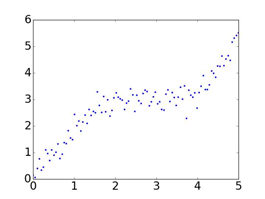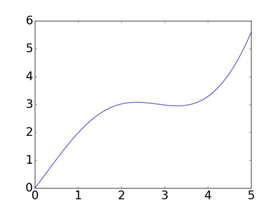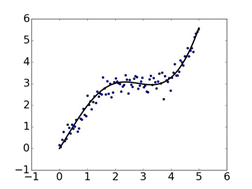基本的画图方法就是这么简单，如果想了解更多pyplot的属性和方法来画出风格多样的图像，可以参考官网：   pyplot - Matplotlib 1.5.3 documentation http://link.zhihu.com/?target=http%3A//matplotlib.org/users/customizing.html  点和线图表只是最基本的用法，有的时候我们获取了分组数据要做对比，柱状或饼状类型的图或许更合适： import numpy as np import matplotlib as mpl import matplotlib.pyplot as plt   mpl.rcParams['axes.titlesize'] = 20 mpl.rcParams['xtick.labelsize'] = 16 mpl.rcParams['ytick.labelsize'] = 16 mpl.rcParams['axes.labelsize'] = 16 mpl.rcParams['xtick.major.size'] = 0 mpl.rcParams['ytick.major.size'] = 0   # 包含了狗，猫和猎豹的最高奔跑速度，还有对应的可视化颜色 speed_map = {     'dog': (48, '#7199cf'),     'cat': (45, '#4fc4aa'),     'cheetah': (120, '#e1a7a2') } # 整体图的标题 fig = plt.figure('Bar chart & Pie chart') # 在整张图上加入一个子图，121的意思是在一个1行2列的子图中的第一张 ax = fig.add_subplot(121) ax.set_title('Running speed - bar chart') # 生成x轴每个元素的位置 xticks = np.arange(3) # 定义柱状图每个柱的宽度 bar_width = 0.5   # 动物名称 animals = speed_map.keys() # 奔跑速度 speeds = [x for x in speed_map.values()] # 对应颜色 colors = [x for x in speed_map.values()]   # 画柱状图，横轴是动物标签的位置，纵轴是速度，定义柱的宽度，同时设置柱的边缘为透明 bars = ax.bar(xticks, speeds, width=bar_width, edgecolor='none')   # 设置y轴的标题 ax.set_ylabel('Speed(km/h)')   # x轴每个标签的具体位置，设置为每个柱的中央 ax.set_xticks(xticks+bar_width/2) # 设置每个标签的名字 ax.set_xticklabels(animals)   # 设置x轴的范围 ax.set_xlim([bar_width/2-0.5, 3-bar_width/2]) # 设置y轴的范围 ax.set_ylim([0, 125])    # 给每个bar分配指定的颜色  for bar, color in zip(bars, colors):     bar.set_color(color) # 在122位置加入新的图 ax = fig.add_subplot(122) ax.set_title('Running speed - pie chart')   # 生成同时包含名称和速度的标签 labels = ['{}\n{} km/h'.format(animal, speed) for animal, speed in zip(animals, speeds)] # 画饼状图，并指定标签和对应颜色 ax.pie(speeds, labels=labels, colors=colors) plt.show()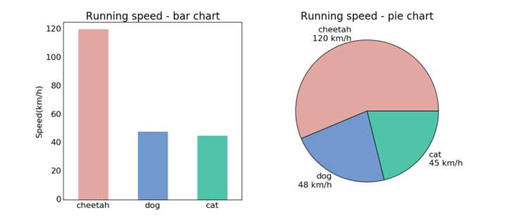在这段代码中又出现了一个新的东西叫做，一个用ax命名的对象。在Matplotlib中，画图时有两个常用概念，一个是平时画图蹦出的一个窗口，这叫一个figure。Figure相当于一个大的画布，在每个figure中，又可以存在多个子图，这种子图叫做axes。顾名思义，有了横纵轴就是一幅简单的图表。在上面代码中，先把figure定义成了一个一行两列的大画布，然后通过fig.add_subplot()加入两个新的子图。subplot的定义格式很有趣，数字的前两位分别定义行数和列数，最后一位定义新加入子图的所处顺序，当然想写明确些也没问题，用逗号分开即可。  5.3.1 3D图表 Matplotlib中也能支持一些基础的3D图表，比如曲面图，散点图和柱状图。这些3D图表需要使用mpl_toolkits模块，先来看一个简单的曲面图的例子：   import matplotlib.pyplot as plt import numpy as np # 3D图标必须的模块，project='3d'的定义 from mpl_toolkits.mplot3d import Axes3D       np.random.seed(42) n_grids = 51             # x-y平面的格点数 c = n_grids / 2             # 中心位置 nf = 2                 # 低频成分的个数 # 生成格点 x = np.linspace(0, 1, n_grids) y = np.linspace(0, 1, n_grids)   # x和y是长度为n_grids的array # meshgrid会把x和y组合成n_grids*n_grids的array，X和Y对应位置就是所有格点的坐标 X, Y = np.meshgrid(x, y)   # 生成一个0值的傅里叶谱 spectrum = np.zeros((n_grids, n_grids), dtype=np.complex) # 生成一段噪音，长度是(2*nf+1)**2/2 noise = [np.complex(x, y) for x, y in np.random.uniform(-1,1,((2*nf+1)**2/2, 2))] # 傅里叶频谱的每一项和其共轭关于中心对称 noisy_block = np.concatenate((noise, [0j], np.conjugate(noise[::-1])))   # 将生成的频谱作为低频成分 spectrum[c-nf:c+nf+1, c-nf:c+nf+1] = noisy_block.reshape((2*nf+1, 2*nf+1))   # 进行反傅里叶变换 Z = np.real(np.fft.ifft2(np.fft.ifftshift(spectrum)))   # 创建图表 fig = plt.figure('3D surface & wire')   # 第一个子图，surface图 ax = fig.add_subplot(1, 2, 1, projection='3d')   # alpha定义透明度，cmap是color map # rstride和cstride是两个方向上的采样，越小越精细，lw是线宽 ax.plot_surface(X, Y, Z, alpha=0.7, cmap='jet', rstride=1, cstride=1, lw=0)   # 第二个子图，网线图 ax = fig.add_subplot(1, 2, 2, projection='3d') ax.plot_wireframe(X, Y, Z, rstride=3, cstride=3, lw=0.5)   plt.show() 这个例子中先生成一个所有值均为0的复数array作为初始频谱，然后把频谱中央部分用随机生成，但同时共轭关于中心对称的子矩阵进行填充。这相当于只有低频成分的一个随机频谱。最后进行反傅里叶变换就得到一个随机波动的曲面，图像如下：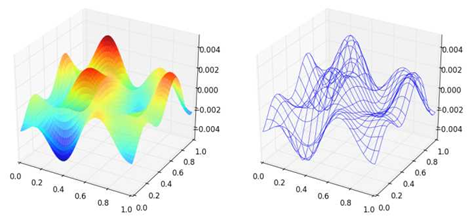3D的散点图也是常常用来查看空间样本分布的一种手段，并且画起来比表面图和网线图更加简单，来看例子： import matplotlib.pyplot as plt import numpy as np from mpl_toolkits.mplot3d import Axes3D   np.random.seed(42) # 采样个数500 n_samples = 500 dim = 3   # 先生成一组3维正态分布数据，数据方向完全随机 samples = np.random.multivariate_normal(     np.zeros(dim),     np.eye(dim),     n_samples )   # 通过把每个样本到原点距离和均匀分布吻合得到球体内均匀分布的样本 for i in range(samples.shape):     r = np.power(np.random.random(), 1.0/3.0)     samples[i] *= r / np.linalg.norm(samples[i])   upper_samples = [] lower_samples = []   for x, y, z in samples:     # 3x+2y-z=1作为判别平面     if z > 3*x + 2*y - 1:         upper_samples.append((x, y, z))     else:         lower_samples.append((x, y, z))   fig = plt.figure('3D scatter plot') ax = fig.add_subplot(111, projection='3d')   uppers = np.array(upper_samples) lowers = np.array(lower_samples)   # 用不同颜色不同形状的图标表示平面上下的样本 # 判别平面上半部分为红色圆点，下半部分为绿色三角 ax.scatter(uppers[:, 0], uppers[:, 1], uppers[:, 2], c='r', marker='o') ax.scatter(lowers[:, 0], lowers[:, 1], lowers[:, 2], c='g', marker='^')   plt.show() 这个例子中，为了方便，直接先采样了一堆3维的正态分布样本，保证方向上的均匀性。然后归一化，让每个样本到原点的距离为1，相当于得到了一个均匀分布在球面上的样本。再接着把每个样本都乘上一个均匀分布随机数的开3次方，这样就得到了在球体内均匀分布的样本，最后根据判别平面3x+2y-z-1=0对平面两侧样本用不同的形状和颜色画出，图像如下：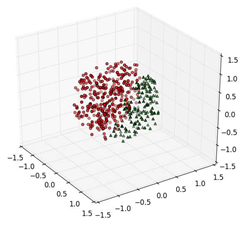5.3.1 图像显示 Matplotlib也支持图像的存取和显示，并且和OpenCV一类的接口比起来，对于一般的二维矩阵的可视化要方便很多，来看例子： import matplotlib.pyplot as plt   # 读取一张小白狗的照片并显示 plt.figure('A Little White Dog') little_dog_img = plt.imread('little_white_dog.jpg') plt.imshow(little_dog_img)   # Z是上小节生成的随机图案，img0就是Z，img1是Z做了个简单的变换 img0 = Z img1 = 3*Z + 4   # cmap指定为'gray'用来显示灰度图 fig = plt.figure('Auto Normalized Visualization') ax0 = fig.add_subplot(121) ax0.imshow(img0, cmap='gray')   ax1 = fig.add_subplot(122) ax1.imshow(img1, cmap='gray')   plt.show() 这段代码中第一个例子是读取一个本地图片并显示，第二个例子中直接把上小节中反傅里叶变换生成的矩阵作为图像拿过来，原图和经过乘以3再加4变换的图直接绘制了两个形状一样，但是值的范围不一样的图案。显示的时候imshow会自动进行归一化，把最亮的值显示为纯白，最暗的值显示为纯黑。这是一种非常方便的设定，尤其是查看深度学习中某个卷积层的响应图时。得到图像如下：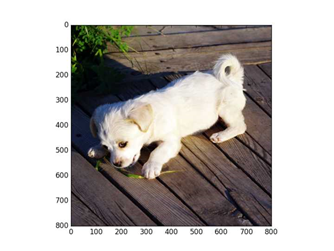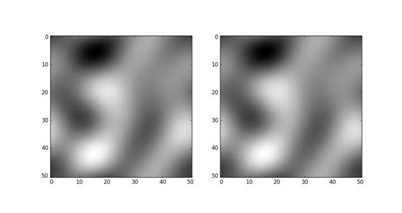只讲到了最基本和常用的图表及最简单的例子，更多有趣精美的例子可以在Matplotlib的官网找到： Thumbnail gallery - Matplotlib 1.5.3 documentation 上篇：给深度学习入门者的Python快速教程 - 基础篇 
展开全文• （还在更新中…） 这篇博客花费了我的大量时间和精力，从创作到维护；若认可本篇博客，希望给一个点赞、收藏 并且，遇到了什么问题，请在评论区留言，我会及时回复的 这本书对Python的知识点的描述很详细，而且排版...
（还在更新中…） 这篇博客花费了我的大量时间和精力，从创作到维护；若认可本篇博客，希望给一个点赞、收藏
并且，遇到了什么问题，请在评论区留言，我会及时回复的

这本书对Python的知识点的描述很详细，而且排版看的很舒服

几个例题： 假装自己从零开始学，将一些有代表性、有意思的例题抽取出来部分复习题： 遇到有意思的复习题，我会拿出来，并且进行分析上机实践： 全部上机实践题的解题思路

文章目录
第一章 Python概述几个例题
第二章 Python语言基础选择题：1、3、7、8思考题：9上机实践：2~6案例研究：使用Pillow库处理图像文件
第三章 程序流程控制几个例题选择题：1、2、3填空题：6思考题：3~6上机实践：2~14案例研究：使用嵌套循环实现图像处理算法
第四章 常用内置数据类型几个例题选择题：11填空题：4、7、8、9、10、13、21思考题：5上机实践：2~14案例研究：科学计算和数据分析
第五章 序列数据类型几个例题选择题：4、5、7、11、12填空题：1、5、6、12、13、14思考题：2、3、5上机实践：2~6案例研究：猜单词游戏
第六章 输入和输出几个例题填空题：1、2例题及上机实践：2~5案例研究：21点扑克牌游戏
第七章 错误和异常处理几个例题案例研究：使用调试器调试Python程序
第八章 函数和函数式编程一些知识点总结和几个例题选择题：1~5思考题：4~11上机实践：2~5案例研究：井字棋游戏
第九章 面向对象的程序设计第十章 模块和客户端第十一章 算法与数据结构基础第十二章 图形用户界面案例研究：简易图形用户界面计算器
第十三章 图形绘制图形绘制模块：tkinter图形绘制模块：turtle
第十四章 数值日期和时间处理第十五章 字符串和文本处理第十六章 文件和数据交换第十七章 数据访问第十八章 网络编程和通信第十九章 并行计算：进程、线程和协程第二十章 系统管理

第一章 Python概述

几个例题
一：Python3.7.4下载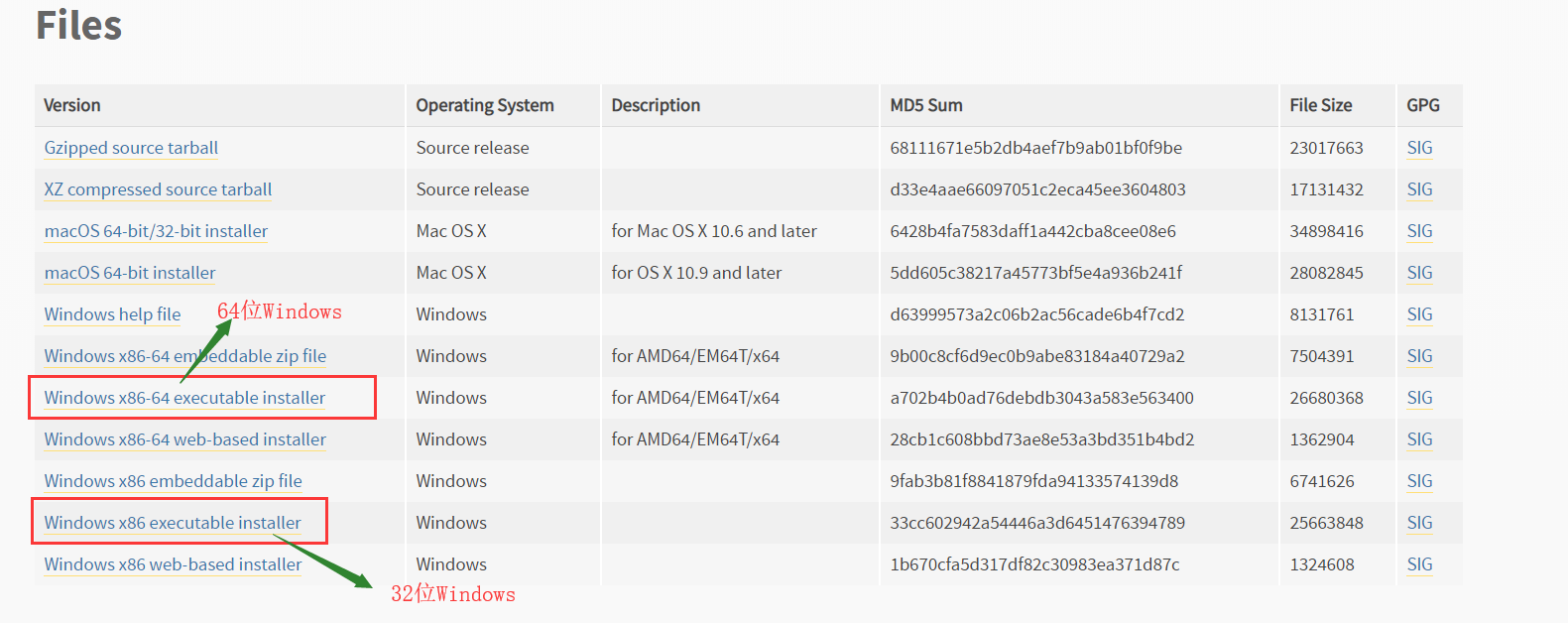下载，安装完python后：出现的四个玩意：Python 3.7 Module Docs，IDLE，Python 3.7 Manuals，Python 3.7（64-bit）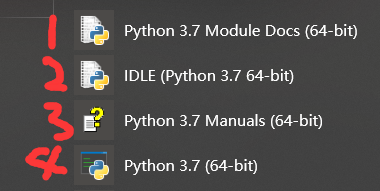Python 3.7 Module Docs（64-bit） 点击之后，会出现一个网页（将我下载的Python3.7.4文件夹中包含的模块都列了出来,页面不止这么点，还可以往下拉）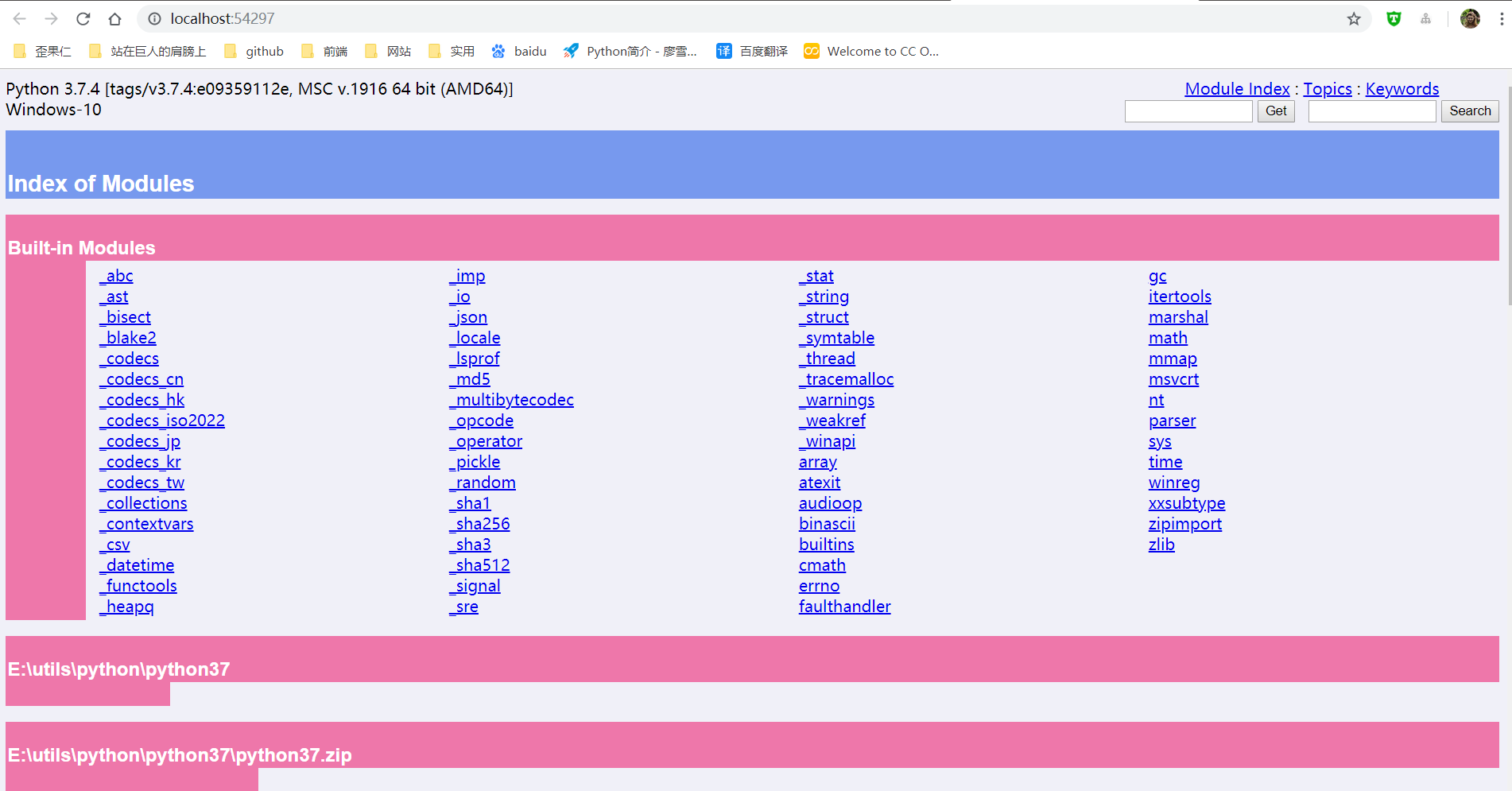IDLE（Python 3.7 64-bit） 一个Python编辑器，Python内置的集成开发工具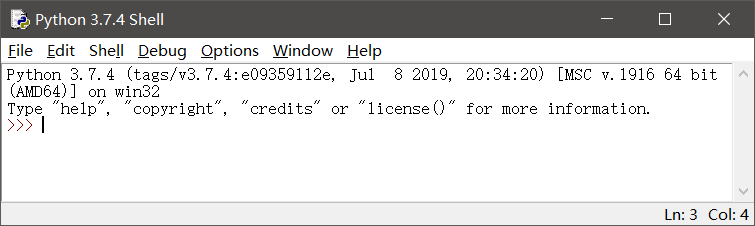Python 3.7 Manuals（64-bit） Python 3.7 开发手册  Python 3.7（64-bit） 控制台中运行Python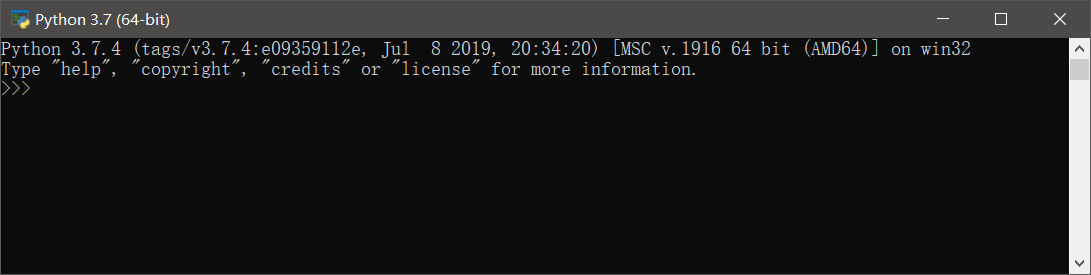二：更新pip和setuptools包，安装NumPy包，安装Matplotlib包
以下三个命令都是在控制台（windows中的cmd）中运行
更新pip和setuptools包
pip用于安装和管理Python扩展包setuptools用于发布Python包
python -m pip install -U pip setuptools

安装NumPy
Python扩展模块NumPy提供了数组和矩阵处理，以及傅立叶变换等高效的数值处理功能
 python -m pip install NumPy

安装Matplotlib包
Matplotlib是Python最著名的绘图库之一，提供了一整套和MATLAB相似的命令API，既适合交互式地进行制图，也可以作为绘图控件方便地嵌入到GUI应用程序中
python -m pip install Matplotlib

三：使用IDLE打开和执行Python源文件程序
首先： 有一个.py文件test.py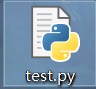使用IDLE打开.py文件的两种方式：
右键test.py---->Edit With IDLE---->Edit With IDLE 3.7（64-bit）打开IDLE，然后File---->Open（或者ctrl+O）选择.py文件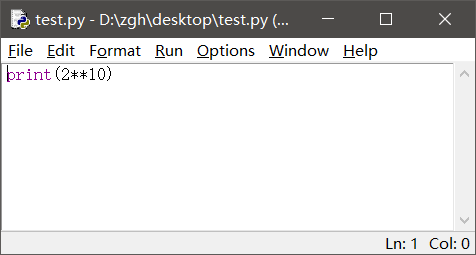运行
Run---->Run Module（或者F5） 就会出现这个界面，执行结果显示在这个界面中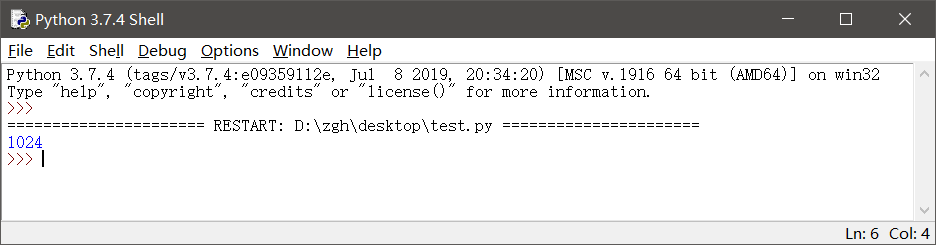补充一点： 如果在IDLE中编辑.py文件，记得修改后要保存（ctrl+s），再运行（F5）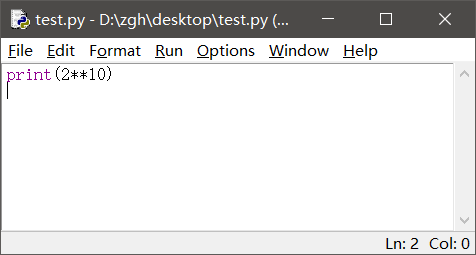四：使用资源管理器运行hello.py
hello.py文件在桌面
import random

print("hello,Python")
print("你今天的随机数字是：",random.choice(range(10)))#输出在0-9之间随机选择的整数
input()

在桌面打开PowerShell（还有两种输入方式：python hello.py或者.\hello.py）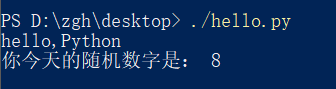或者在桌面打开cmd, 就输入hello.py或者python hello.py
补充：上述两种命令中的hello.py都是相对路径，因为文件在桌面，而且我是在桌面打开cmd，所以文件路劲可以这么简简单单的写。如果文件存储位置和cmd打开位置不一样，请使用绝对路径
五：命令行参数示例hello_argv.py
hello_argv.py文件在桌面
import sys

print("Hello,",sys.argv)
#这样写也行：
#print("Hello,"+sys.argv)

在桌面打开PowerShell（还有两种输入方式：python hello_argv.py 任意输入或者./hello_argv.py 任意输入）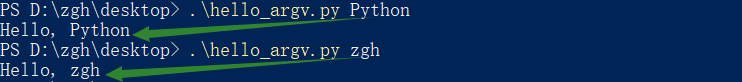或者在桌面打开cmd，就输入hello_argv.py 任意输入或者python hello_argv.py 任意输入
补充：以图中第一个命令举例，hello_argv.py即sys.argv；Python即sys.argv
第二章 Python语言基础

选择题：1、3、7、8
1. 在Python中，以下标识符合法的是
A. _B. 3CC. it’sB. str
答案：A

标识符的第一个字符必须是字母，下划线（_）；其后的字符可以是字母、下划线或数字。一些特殊的名称，作为python语言的保留关键字，不能作为标识符以双下划线开始和结束的名称通常具有特殊的含义。例如__init__为类的构造函数，一般应避免使用

B：以数字开头，错误 C：使用了'，不是字母、下划线或数字 D：str是保留关键字
3. 在下列Python语句中非法的是
A. x = y =1B. x = (y =1)C. x,y = y,xB. x=1;y=1
答案：B，C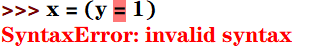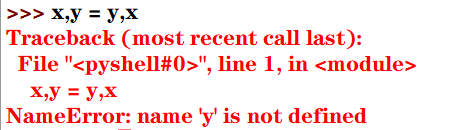7. 为了给整型变量x，y，z赋初值10，下面Python赋值语句正确的是
A. xyz=10B. x=10 y=10 z=10C. x=y=z=10B. x=10，y=10，z=10
答案：C

分号;用于在一行书写多个语句python支持链式赋值

A：赋值对象是xyz B：分号;用于在一行书写多个语句，而不是' '（即空格） D：分号;用于在一行书写多个语句，而不是,
8. 为了给整型变量x，y，z赋初值5，下面Python赋值语句正确的是
A. x=5;y=5;z=5B. xyz=5C. x,y,z=10B. x=10，y=10，z=10
答案：A

Pytho能支持序列解包赋值，但是变量的个数必须与序列的元素个数一致，否则会报错

B：赋值对象是xyz C：序列解包赋值，变量的个数必须与序列的元素个数一致，否则会报错 D：分号;用于在一行书写多个语句，而不是,
思考题：9
9.下列Python语句的输出结果是
def f():pass
print(type(f()))

结果：<class 'NoneType'>

NoneType数据类型包含唯一值None，主要用于表示空值，如没有返回值的函数的结果

上机实践：2~6
2. 编写程序，输入本金、年利率和年数，计算复利（结果保留两位小数）
money = int(input("请输入本金："))
rate = float(input("请输入年利率："))
years = int(input("请输入年数："))
amount = money*((1+rate/100)**years)
print(str.format("本金利率和为：{0:2.2f}",amount))

运行：
请输入本金：1000
请输入年利率：6.6
请输入年数：10
本金利率和为：1894.84

3. 编写程序，输入球的半径，计算球的表面积和体积（结果保留两位小数）
import math
r = float(input("请输入球的半径："))
area = 4 * math.pi * r**2
volume = 4/3*math.pi*r**3
print(str.format("球的表面积为：{0:2.2f}，体积为：{1:2.2f}",area,volume))

运行：
请输入球的半径：666
球的表面积为：5573889.08，体积为：1237403376.70

4. 编写程序，声明函数getValue(b,r,n)，根据本金b，年利率r和年数n计算最终收益v
money = int(input("请输入本金："))
rate = float(input("请输入年利率(<1)："))
years = int(input("请输入年数："))

def getValue(b,r,n):
return b*(1+r)**n

print(str.format("本金利率和为：{0:2.2f}",getValue(money,rate,years)))

运行：
请输入本金：10000
请输入年利率(<1)：0.6
请输入年数：6
本金利率和为：167772.16

5. 编写程序，求解一元二次方程x2-10x+16=0
from math import sqrt
x = (10+sqrt(10*10-4*16))/2
y = (10-sqrt(10*10-4*16))/2
print(str.format("x*x-10*x+16=0的解为：{0:2.2f}，{1:2.2f}",x,y))

运行：
x*x-10*x+16=0的解为：8.00，2.00

6. 编写程序，提示输入姓名和出生年份，输出姓名和年龄
import datetime
sName = str(input("请输入您的姓名："))
birthday = int(input("请输入您的出生年份："))
age = datetime.date.today().year - birthday
print("您好！{0}。您{1}岁。".format(sName,age))

运行：
请输入您的姓名：zgh
请输入您的出生年份：1999
您好！zgh。您20岁。

案例研究：使用Pillow库处理图像文件
https://blog.csdn.net/Zhangguohao666/article/details/102060722
通过此案例，进一步了解Python的基本概念：模块、对象、方法和函数的使用
第三章 程序流程控制

几个例题
一：编程判断某一年是否为闰年

闰年：年份能被4整除但不能被100整除，或者可以被400整除。 口诀：四年一闰，百年不闰，四百必闰

代码一：
y = int(input("请输入要判断的年份："))
if((y % 4 == 0 and y % 100 != 0) or y % 400 == 0):
print("是闰年")
else:
print("不是闰年")

代码二（使用calendar模块的isleap()函数来判断）：
from calendar import isleap

y = int(input("请输入要判断的年份："))
if(isleap(y)):print("闰年")
else:print("不是闰年")

二：利用嵌套循环打印九九乘法表
九九乘法表：
for i in range(1,10):
s = ""
for j in range(1,10):
s += str.format("%d * %d = %02d  " %(i, j, i*j))
print(s)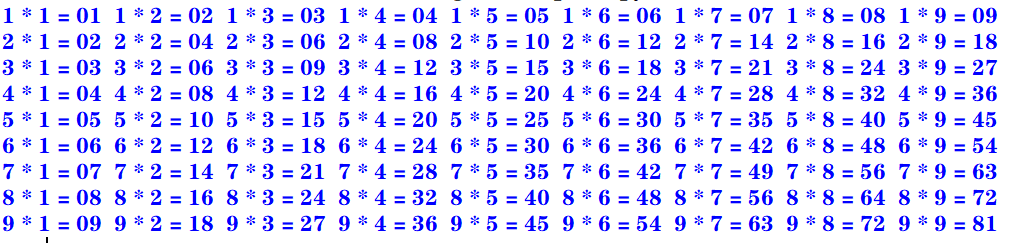下三角：
for i in range(1,10):
s = ""
for j in range(1,i+1):
s += str.format("%d * %d = %02d  " %(i, j, i*j))
print(s)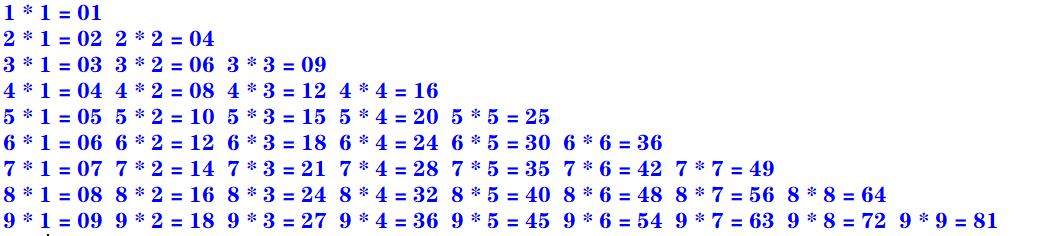上三角：
for i in range(1,10):
s = ""
for k in range(1,i):
s += "                   "
for j in range(i,10):
s += str.format("%d * %d = %02d  " %(i, j, i*j))
print(s)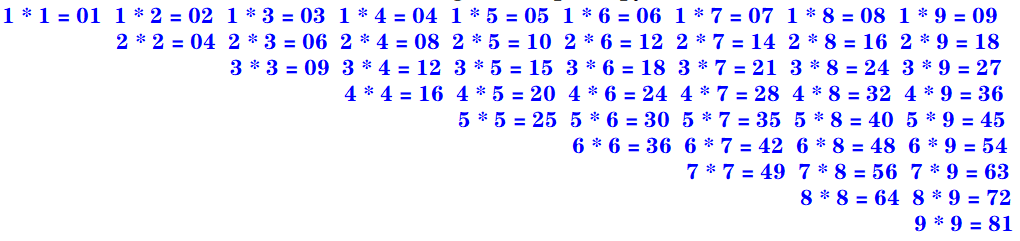三：enumerate()函数和下标元素循环示例

Python语言中的for循环直接迭代对象集合中的元素，如果需要在循环中使用索引下标访问集合元素，则可以使用内置的enumerate()函数

enumerate()函数用于将一个可遍历的数据对象（例如列表、元组或字符串）组合为一个索引序列，并返回一个可迭代对象，故在for循环当中可直接迭代下标和元素
seasons = ["Spring","Summer","Autumn","Winter"]
for i,s in enumerate(seasons,start=1):    #start默认从0开始
print("第{0}个季节：{1}".format(i,s))

运行：
第1个季节：Spring
第2个季节：Summer
第3个季节：Autumn
第4个季节：Winter

四：zip()函数和并行循环示例

如果需要并行遍历多个可迭代对象，则可以使用Python的内置函数zip()

zip()函数将多个可迭代对象中对应的元素打包成一个个元组，然后返回一个可迭代对象。如果元素的个数不一致，则返回列表的长度与最短的对象相同。
利用运算符*还可以实现将元组解压为列表
evens = [0,2,4,6,8]
odds = [1,3,5,7,9]
for e,o in zip(evens,odds):
print("{0} * {1} = {2}".format(e,o,e*o))

运行：
0 * 1 = 0
2 * 3 = 6
4 * 5 = 20
6 * 7 = 42
8 * 9 = 72

五：map()函数和循环示例

如果需要遍历可迭代对象，并使用指定函数处理对应的元素，则可以使用Python的内置函数map()

map(func,seq1[,seq2,...])

func作用于seq中的每一个元素，并将所有的调用结果作为可迭代对象返回。如果func为None，该函数的作用等同于zip()函数
计算绝对值：
>>> list(map(abs, [-1, 0, 7, -8]))
[1, 0, 7, 8]

计算乘幂：
>>> list(map(pow, range(5), range(5)))
[1, 1, 4, 27, 256]

计算ASCII码：
>>> list(map(ord, 'zgh'))
[122, 103, 104]

字符串拼接（使用了匿名函数lambda）：
>>> list(map(lambda x, y: x+y, 'zgh', '666'))
['z6', 'g6', 'h6']

选择题：1、2、3
1. 下面的Python循环体的执行次数与其他不同的是
A.
i = 0
while(i <= 10):
print(i)
i = i + 1

B.
i = 10
while(i > 0):
print(i)
i = i - 1

C.
for i in range(10):
print(i)

D.
for i in range(10,0,-1):
print(i)

答案：A
A：[0,10] 执行11次 B：[10,1] 执行10次 C：[0,9) 执行10次 D：[10,0) 执行10次
2. 执行下列Python语句将产生的结果是
x = 2; y = 2.0
if(x == y): print("Equal")
else: print("Not Equal")

A. EqualB. Not EqualC. 编译错误D. 运行时错误
答案：A

Python中的自动类型转换：
自动类型转换注意针对Number数据类型来说的当2个不同类型的数据进行运算的时候,默认向更高精度转换数据类型精度从低到高:bool int float complex关于bool类型的两个值：True 转化成整型是1；False 转化成整型是0

int类型的2转化为float类型的2.0
3. 执行下列Python语句将产生的结果是
i= 1
if(i): print(True)
else: print(False)

A. 输出1B. 输出TrueC. 输出FalseD. 编译错误
答案：B
在Python中，条件表达式最后被评价为bool值True或False。
如果表达式的结果为数值类型（0），空字符串（""），空元组（()），空列表（[]），空字典（{}），其bool值为False，否则其bool值为True
填空题：6
6. 要使语句for i in range(_,-4,-2)循环执行15次，则循环变量i的初值应当为
答案：26或者25
一开始我给的答案是26，经过评论区 亻的提醒：>>> a = 0
>>> for i in range(26, -4, -2): a+=1

>>> print(a)
15

>>> a = 0
>>> for i in range(25, -4, -2): a+=1

>>> print(a)
15

这种题目有一个规律：for i in range(x,y,z): 若循环中没有break或者continue语句， 执行次数的绝对值：result = (x-y)÷z
但实际上没有这么简单：
如果步长为 -1或者1，那么答案只有一个如果步长为 -2或者2，那么答案有两个如果步长为 -3或者3，那么答案有三个…
通过公式算出 x 之后，
如果步长为2，还要计算 (x ± 1) - z × (result-1) 的值，然后再经过琐碎的判断即可如果步长为3，还要计算 (x ± 2) - z × (result-1) 的值，……
虽然看着麻烦，但实际上是很好理解的
思考题：3~6
3. 阅读下面的Python程序，请问程序的功能是什么？
from math import sqrt

n = 0
for m in range(101,201,2):
k = int(sqrt(m))
for i in range(2, k+2):
if m % i == 0:break
if i == k + 1:
if n % 10 == 0:print()
print('%d' % m,end = " ")
n += 1

输出101到200之间的素数 每行输出10个，多余换行
运行：
101 103 107 109 113 127 131 137 139 149
151 157 163 167 173 179 181 191 193 197
199


素数（质数）是指在大于1的自然数中，除了1和它本身以外不再有其他因数的自然数。

4. 阅读下面的Python程序，请问输出的结果使什么？
n = int(input("请输入图形的行数："))
for i in range(0, n):
for j in range(0, 10 - i):print(" ", end=" ")
for k in range(0, 2 * i + 1):print(" * ", end=" ")
print("\n")

输出的是一个金字塔
运行：
请输入图形的行数：4
*

*   *   *

*   *   *   *   *

*   *   *   *   *   *   *

5. 阅读下面的Python程序，请问输出的结果使什么？程序的功能是什么？
for i in range(100,1000):
n1 = i // 100
n2 = i // 10 % 10
n3 = i % 10
if(pow(n1, 3) + pow(n2, 3) + pow(n3, 3) == i):print(i, end=" ")

输出三位数中所有的水仙花数
运行：
153 370 371 407


水仙花数 是指一个 3 位数，它的每个位上的数字的 3次幂之和等于它本身

6. 阅读下面的Python程序，请问输出的结果使什么？程序的功能是什么？
for n in range(1,1001):
total = 0; factors = []
for i in range(1, n):
if(n % i == 0):
factors.append(i)
total += i
if(total == n):print("{0} : {1}".format(n, factors))

输出1到1000的所有完数，并输出每个完数的所有因子
运行：
6 : [1, 2, 3]
28 : [1, 2, 4, 7, 14]
496 : [1, 2, 4, 8, 16, 31, 62, 124, 248]


完数 所有的真因子（即除了自身以外的约数）的和（即因子函数），恰好等于它本身

上机实践：2~14
2. 编写程序，计算1=2+3+…+100之和
使用for循环（递增）：
total = 0
for i in range(101):
total += i
print(total)

使用求和公式：
>>> (1 + 100) * 100 /2
5050.0

使用累计迭代器itertools.accumulate
>>> import itertools
>>> list(itertools.accumulate(range(1, 101)))
5050

3. 编写程序，计算10+9+8+…+1之和
使用for循环（递增）：
total = 0
for i in range(11):
total += i
print(total)

使用for循环（递减）：
total = 0
for i in range(10,0,-1):
total += i
print(total)

使用求和公式：
>>> (1 + 10) * 10 / 2
55.0

使用累计迭代器itertools.accumulate
>>> import itertools
>>> list(itertools.accumulate(range(1,11)))
55

4. 编写程序，计算1+3+5+7+…+99之和
使用for循环（递增）：
total = 0
for i in range(1,100,2):
total += i
print(total)

使用求和公式：
>>> (1 + 99) * 50 /2
2500.0

使用累计迭代器itertools.accumulate
>>> import itertools
>>> list(itertools.accumulate(range(1,100,2)))
2500

5. 编写程序，计算2+4+6+8+…+100之和
使用for循环（递增）：
total = 0
for i in range(2,101,2):
total += i
print(total)

使用求和公式：
>>> (2 + 100) * 50 / 2
2550.0

使用累计迭代器itertools.accumulate
>>> import itertools
>>> x = list(itertools.accumulate(range(2,101,2)))
>>> x[len(x)-1]
2550

6. 编写程序，使用不同的实现方法输出2000~3000的所有闰年
代码一：
for y in range(2000,3001):
if((y % 4 == 0 and y % 100 != 0) or y % 400 == 0):
print(y,end = ' ')

代码二（使用calendar模块的isleap()函数来判断）：
from calendar import isleap

for y in range(2000,3001):
if(isleap(y)):print(y,end = " ")

运行：
2000 2004 2008 2012 2016 2020 2024 2028 2032 2036 2040 2044 2048 2052 2056 2060 2064 2068 2072 2076 2080 2084 2088 2092 2096 2104 2108 2112 2116 2120 2124 2128 2132 2136 2140 2144 2148 2152 2156 2160 2164 2168 2172 2176 2180 2184 2188 2192 2196 2204 2208 2212 2216 2220 2224 2228 2232 2236 2240 2244 2248 2252 2256 2260 2264 2268 2272 2276 2280 2284 2288 2292 2296 2304 2308 2312 2316 2320 2324 2328 2332 2336 2340 2344 2348 2352 2356 2360 2364 2368 2372 2376 2380 2384 2388 2392 2396 2400 2404 2408 2412 2416 2420 2424 2428 2432 2436 2440 2444 2448 2452 2456 2460 2464 2468 2472 2476 2480 2484 2488 2492 2496 2504 2508 2512 2516 2520 2524 2528 2532 2536 2540 2544 2548 2552 2556 2560 2564 2568 2572 2576 2580 2584 2588 2592 2596 2604 2608 2612 2616 2620 2624 2628 2632 2636 2640 2644 2648 2652 2656 2660 2664 2668 2672 2676 2680 2684 2688 2692 2696 2704 2708 2712 2716 2720 2724 2728 2732 2736 2740 2744 2748 2752 2756 2760 2764 2768 2772 2776 2780 2784 2788 2792 2796 2800 2804 2808 2812 2816 2820 2824 2828 2832 2836 2840 2844 2848 2852 2856 2860 2864 2868 2872 2876 2880 2884 2888 2892 2896 2904 2908 2912 2916 2920 2924 2928 2932 2936 2940 2944 2948 2952 2956 2960 2964 2968 2972 2976 2980 2984 2988 2992 2996

7. 编写程序，计算Sn=1-3+5-7+9-11…
代码一：
n = int(input("项数："))
total = 0
flag = True
for i in range(1,2*n,2):
if(flag):
total += i
flag = False
else:
total -= i
flag = True
print(total)

代码二：
n = int(input("项数："))
total = 0
x = 2
for i in range(1,2*n,2):
total += pow(-1,x)*i
x += 1
print(total)

运行：
项数：10
-10

8. 编写程序，计算Sn=1+1/2+1/3+…
n = int(input("项数："))
total = 0.0
for i in range(1,n+1):
total += 1/i
print(total)

运行：
项数：10
2.9289682539682538

9. 编写程序，打印九九乘法表。要求输入九九乘法表的各种显示效果（上三角，下三角，矩形块等方式）
矩形块：
for i in range(1,10):
s = ""
for j in range(1,10):
s += str.format("%d * %d = %02d  " %(i, j, i*j))
print(s)下三角：
for i in range(1,10):
s = ""
for j in range(1,i+1):
s += str.format("%d * %d = %02d  " %(i, j, i*j))
print(s)上三角：
for i in range(1,10):
s = ""
for k in range(1,i):
s += "                   "
for j in range(i,10):
s += str.format("%d * %d = %02d  " %(i, j, i*j))
print(s)10. 编写程序，输入三角形的三条边，先判断是否可以构成三角形，如果可以，则进一步求三角形的周长和面积，否则报错“无法构成三角形！”
from math import sqrt

a = float(input("请输入三角形的边长a："))
b = float(input("请输入三角形的边长b："))
c = float(input("请输入三角形的边长c："))

if(a < b): a,b = b,a
if(a < c): a,c = c,a
if(b < c): b,c = c,b

if(a < 0 or b < 0 or c < 0 or b+c <= a): print("无法构成三角形！")
else:
h = (a+b+c)/2
area = sqrt(h*(h-a)*(h-b)*(h-c))
print("周长：{0}，面积：{1}".format(a+b+c,area))

运行：
请输入三角形的边长a：4
请输入三角形的边长b：3
请输入三角形的边长c：5
周长：12.0，面积：6.0

11. 编写程序，输入x，根据如下公式计算分段函数y的值。请分别用单分支语句，双分支语句结构以及条件运算语句等方法实现
y = (x2-3x)/(x+1) + 2π + sinx （x≥0 ） y = ln(-5x) + 6√(|x|+e4) - (x+1)3 (x<0)
单分支语句：
import math

x = float(input("请输入x："))
if(x >= 0):
y = (x*x - 3*x)/(x+1) + 2*math.pi + math.sin(x)
if(x < 0):
y = math.log(-5*x) + 6 * math.sqrt(abs(x) + math.exp(4)) - pow(x+1,3)

print(y)


双分支语句：
import math

x = float(input("请输入x："))
if(x >= 0):
y = (x*x - 3*x)/(x+1) + 2*math.pi + math.sin(x)
else:
y = math.log(-5*x) + 6 * math.sqrt(abs(x) + math.exp(4)) - pow(x+1,3)

print(y)

条件运算语句：
import math

x = float(input("请输入x："))
y = ((x*x - 3*x)/(x+1) + 2*math.pi + math.sin(x)) if(x >= 0) \
else (math.log(-5*x) + 6 * math.sqrt(abs(x) + math.exp(4)) - pow(x+1,3))

print(y)

运行一：
请输入x：666
668.2715406628656

运行二：
请输入x：-666
294079794.1744833

12. 编写程序，输入一元二次方程的3个系数a、b、c，求ax2+bx+c=0方程的解
import math

a = float(input("请输入系数a："))
b = float(input("请输入系数b："))
c = float(input("请输入系数c："))

delta = b*b -4*a*c

if(a == 0):
if(b == 0): print("无解")
else: print("有一个实根：",-1*c/b)
elif(delta == 0): print("有两个相等实根：x1 = x2 = ", (-1*b)/(2*a))
elif(delta > 0): print("有两个不等实根：x1 = {0}，x2 = {1}".format\
((-1*b +math.sqrt(delta))/2*a,(-1*b -math.sqrt(delta))/2*a))
elif(delta < 0): print("有两个共轭复根：x1 = {0}，x2 = {1}".format\
(complex( (-1*b)/(2*a),math.sqrt(delta*-1)/(2*a)),complex( (-1*b)/(2*a),-1*math.sqrt(delta*-1)/(2*a))))

运行一：
请输入系数a：0
请输入系数b：0
请输入系数c：10
无解

运行二：
请输入系数a：0
请输入系数b：10
请输入系数c：5
有一个实根： -0.5

运行三：
请输入系数a：1
请输入系数b：8
请输入系数c：16
有两个相等实根：x1 = x2 =  -4.0

运行四：
请输入系数a：1
请输入系数b：-5
请输入系数c：6
有两个不等实根：x1 = 3.0，x2 = 2.0

运行五：
请输入系数a：5
请输入系数b：2
请输入系数c：1
有两个共轭复根：x1 = (-0.2+0.4j)，x2 = (-0.2-0.4j)

13. 编写程序，输入整数n（n≥0）,分别利用for循环和while循环求n!
for循环
n = int(input("请输入n："))

if(n == 0): total = 1
if(n > 0):
total = 1
for i in range(n,0,-1):
total *= i

print(total)


while循环
n = int(input("请输入n："))

if(n == 0): total = 1
if(n > 0):
total = 1
while(n >= 1):
total *= n
n -= 1

print(total)

补充一个：使用累计迭代器itertools.accumulate
>>> import itertools, operator
>>> n = int(input('请输入n：'))
请输入n：7
>>> x = list(accumulate(range(1, n+1), operator.mul))
>>> x[len(x)-1]
5040

14. 编写程序，产生两个0~100（包含0和100）的随机整数a和b，求这两个整数的最大公约数和最小公倍数
现有知识点解决方法

import random

a = random.randint(0,100)
b = random.randint(0,100)
sum = a*b

print(a) #输出原来的a，b
print(b)

if(a < b): a,b = b,a

while(a%b != 0):
a,b = b,a%b

print("最大公约数：{0}，最小公倍数：{1}".format(b,sum/b))


补充：使用生成器（generate）函数：yield
>>> def func(a, b):
if(a < b): a,b = b,a
while(a%b != 0):
a,b = b,a%b
yield b

>>> import random
>>> if __name__ == '__main__':
a = random.randint(0,100)
b = random.randint(0,100)
sum = a*b
print(a,b)
t = list(iter(func(a, b)))
gcd = t[len(t)-1]
print("gcd = {0}, mcm = {1}".format(gcd, sum/gcd))

29 65
gcd = 1, mcm = 1885.0

补充：使用math模块中的gcd(x,y)函数
>>> import random
>>> import math
>>> if __name__ == '__main__':
a = random.randint(0,100)
b = random.randint(0,100)
sum = a*b
print(a,b)
gcd = math.gcd(a,b)
print("gcd = {0}, mcm = {1}".format(gcd, sum/gcd))

29 48
gcd = 1, mcm = 1392.0

案例研究：使用嵌套循环实现图像处理算法
https://blog.csdn.net/Zhangguohao666/article/details/103935185
通过图像处理算法案例，深入了解Python数据结构和基本算法流程
第四章 常用内置数据类型

几个例题
一：Python内置数据类型概述
Python中一切皆为对象，而每个对象属于某个数据类型

Python的数据类型包括：
内置的数据类型模块中定义的数据类型用户自定义的类型

四种内置的数值类型：int，float，bool，complex

int 与其他计算机语言有精度限制不同，Python中的整数位数可以为任意长度（只受限于计算机内存）。 整型对象是不可变对象。float 与其他计算机语言中的double和float对应 Python的浮点类型的精度和系统相关boolcomplex 当数值字符串中包含虚部j（或J）时即复数字面量

序列数据类型：str，tuple，bytes，list，bytearray

序列数据类型表示若干有序数据.

不可变序列数据类型：
str（字符串） 表示Unicode字符序列，例如：“zgh666” 在Python中没有独立的字符数据类型，字符即长度为1的字符串tuple（元组） 表示任意数据类型的序列，例如：(“z”,“g”,“h”,6,6,6)bytes（字节序列） 表示字节（8位）序列数据

可变序列数据类型：
list（列表） 表示可以修改的任意类型数据的序列，比如：[‘z’,‘g’,‘h’,6,6,6]bytearray（字节数组） 表示可以修改的字节（8位）数组

集合数据类型：set，frozenset

集合数据类型表示若干数据的集合，数据项目没有顺序，且不重复
set（集） 例如：{1，2，3}frozenset（不可变集）

字典数据类型：dict

字典数据类型用于表示键值对的字典 例如：{1:"zgh", 2:666}

NoneType，NotImplementedType，EllipsisType

NoneType数据类型包含唯一值None，主要用于表示空值，如没有返回值的函数的结果NotImplementedType数据类型包含唯一值NotImplemented，在进行数值运算和比较运算时，如果对象不支持，则可能返回该值EllipsisType数据类型包含唯一值Ellipsis，表示省略字符串符号...

其他数据类型

Python中一切对象都有一个数据类型，模块、类、对象、函数都属于某种数据类型 Python解释器包含内置类型， 例如： 代码对象Code objects 框架对象Frame objects 跟踪对象Traceback objects 切片对象Slice objects 静态方法对象Static method objects 类方法对象Class method objects

二：整型字面量示例

Python3.7支持使用下划线作为整数或者浮点数的千分位标记，以增强大数值的可阅读性。 二进制、八进制、十六进制则使用下划线区分4位标记

1_000_000_000  #输出1000000000

0xff_ff_ff_ff  #输出4294967295
0x_FF_FF_FF_FF  #输出4294967295

三：字符串字面量示例

两个紧邻的字符串，如果中间只有空格分隔，则自动拼接位一个字符串

'zgh' '666'  #输出'zgh666'
'zgh' + "666"   #输出'zgh666'

四：转义字符示例

转义字符后跟Unicode编码也可以表示字符
\ooo八进制Unicode码对应的字符\xhh十六进制Unicode码对应的字符

'\101'  #输出'A'
'\x41'  #输出'A'


使用r’‘或者R’'的字符串称为原始字符串，其中包含的任何字符都不进行转义

s = r'换\t行\t符\n'
s  		  #输出：'换\\t行\\t符\\n'
print(s)  #输出：换\\t行\\t符\\n

五：字符串的格式化
一：
"student number：{0}，score_average：{1}".format(2,100)
#输出：'student number：2，score_average：100'

二：
str.format("student number：{0}，score_average：{1}",2,100)
#输出：'student number：2，score_average：100'

三（兼容Python2的格式，不推荐使用）：
 "student number：%4d，score_average：%2.1f" %(2,100)
#输出：'student number：   2，score_average：100.0'

六：字符串示例，格式化输出字符串堆积的三角形

str.center()方法用于字符串两边填充str.rjust()方法用于字符串右填充

print("1".center(20))		#一行20个字符，居中对齐
print(format("121","^20"))	#一行20个字符，居中对齐
print("1".rjust(20,"*"))	#一行20个字符，右对齐，加*
print(format("121","*>20"))	#一行20个字符，右对齐，加*

运行：
         1
121
*******************1
*****************121

选择题：11
11. 关于Python字符串，下列说法错误的是
A. 字符即长度为1的字符串 B. 字符串以/0标识字符串的结束 C. 用户既可以用单引号，也可以用双引号创建字符串 D. 用三引号字符串中可以包含换行回车等特殊字符
答案：B

Python中字符串不是用\0来判断字符串结尾， 每个字符串都存有字符串的长度，通过计数来判断是否到达结尾。

虽然在c语言中\0就是来判断字符串的结尾;
填空题：4、7、8、9、10、13、21
4. Python表达式3 ** 2 ** 3的值为
答案：6561

表达式中，相同优先级的运算，从右往左

7. Python语句print(pow(-3,2),round(18.67,1),round(18.67,-1))的输出结果是
答案：9 18.7 20.0

pow()幂运算 round()四舍六入，五留双

8. Python语句print(round(123.84,0),round(123.84,-2),floor(15.5))的输出结果是
答案：124.0 100.0 15

补充：floor()是math模块中的方法，向下取整

9. Python语句print(int(‘20’,16),int(‘101’,2))的输出结果是
答案：32 5

注意：int(x,y)是指将y进制的数值x转化为10进制数

10. Python语句print(hex(16),bin(10))的输出结果是
答案：0x10 0b1010

hex(x)将十进制数x转化为十六进制，以字符串形式输出 bin(x)将十进制数x转化为二进制，以字符串形式输出

13. Python语句print(gcd(12,16),divmod(7,3))的输出结果是
答案：4 (2,1)

gcd()是math模块中的函数，求最大公约数 divmod()是内置函数，返回商和余数

21. Python语句序列 x=True;y=False;z=False;print(x or y and z) 的运行结果是
答案：True

and优先级比or高

思考题：5
5. 阅读下面的Python程序，请问输出结果是什么？
from decimal import *

ctx = getcontext()
ctx.prec = 2
print(Decimal('1.78'))#1.78
print(Decimal('1.78') + 0)#1.8
ctx.rounding = ROUND_UP
print(Decimal('1.65') + 0)#1.7
print(Decimal('1.62') + 0)#1.7
print(Decimal('-1.45') + 0)#-1.5
print(Decimal('-1.42') + 0)#-1.5
ctx.rounding = ROUND_HALF_UP
print(Decimal('1.65') + 0)#1.7
print(Decimal('1.62') + 0)#1.6
print(Decimal('-1.45') + 0)#-1.5
ctx.rounding = ROUND_HALF_DOWN
print(Decimal('1.65') + 0)#1.6
print(Decimal('-1.45') + 0)#-1.4

上机实践：2~14
2. 编写程序，格式化输出杨辉三角

杨辉三角即二项式定理的系数表，各元素满足如下条件：第一列及对角线上的元素均为1；其余每个元素等于它上一行同一列元素与前一列元素之和

我使用了一个更加精妙的规律 比如第一行为1 第二行：01 + 10 = 11 第三行：011 + 110 = 121 第四行：0121 + 1210 = 1331 。。。
def generate(numRows):
l1 = 
n = 0
while n < numRows:
print(str(l1).center(66))
l1 = [sum(t) for t in zip( + l1, l1 + )]  #利用zip函数算出每一行 如第二行 zip([0,1],[1,0])=[1,1],以此类推
n += 1
a=int(input("请输入行数"))
generate(a)

运行：
请输入行数4

[1, 1]
[1, 2, 1]
[1, 3, 3, 1]

3. 输入直角三角形的两个直角边，求三角形的周长和面积，以及两个锐角的度数。结果保留一位小数
import math

a = float(input("请输入直角三角形的直角边a："))
b = float(input("请输入直角三角形的直角边b："))
c = math.sqrt(a*a+b*b)

p = a + b + c
area = 0.5*a*b
print("三角形的周长：{0:1.1f}，面积：{1:1.1f}".format(p,area))

sina = a/c
sinb = b/c

a_degree = round(math.asin(sina) * 180 / math.pi,0)
b_degree = round(math.asin(sinb) * 180 / math.pi,0)

print("三角形直角边a的度数：{0}，b的度数：{1}".format(a_degree,b_degree))

运行：
请输入直角三角形的直角边a：3
请输入直角三角形的直角边b：4
三角形的周长：12.0，面积：6.0
三角形直角边a的度数：37.0，b的度数：53.0

4. 编程产生0~100（包含0和100）的三个随机数a、b、c，要求至少使用两种不同的方法，将三个数按从小到大的顺序排序
方法一：
import random

a = random.randint(0, 100)
b = random.randint(0, 100)
c = random.randint(0, 100)

print(str.format("原始值：{0}，{1}，{2}", a, b, c))

if(a > b): a,b = b,a
if(a > c): a,c = c,a
if(b > c): b,c = c,b

print(str.format("增序：{0}，{1}，{2}", a, b, c))

方法二（使用内置函数max、min、sum）：
import random

a = random.randint(0, 100)
b = random.randint(0, 100)
c = random.randint(0, 100)

print(str.format("原始值：{0}，{1}，{2}", a, b, c))

maxx = max(a, b, c)
minx = min(a, b, c)
median = sum([a, b, c]) - minx - maxx

print(str.format("增序：{0}，{1}，{2}", minx, median, maxx))

方法三（使用内置函数sorted）：
>>> import random
>>> a = random.randint(0,100)
>>> b = random.randint(0,100)
>>> c = random.randint(0,100)
>>> print("init value: {0} , {1} , {2}".format(a,b,c))
init value: 17 , 6 , 59
>>> sorted([a,b,c])
[6, 17, 59]

5. 编程计算有固定工资收入的党员每月所缴纳的党费。
工资基数3000元及以下者，交纳工资基数的0.5% 工资基数3000~5000元者，交纳工资基数的1% 工资基数在5000~10000元者，交纳工资基数的1.5% 工资基数超过10000元者，交纳工资基数的2%
salary = float(input("请输入有固定工资收入的党员的月工资："))
if salary <= 3000: dues = salary*0.005
elif salary <= 5000: dues = salary*0.01
elif salary <= 10000: dues = salary*0.15
else: dues = salary*0.02

print("交纳党费：",dues)

运行：
请输入有固定工资收入的党员的月工资：10001
交纳党费： 200.02

6. 编程实现袖珍计算器，要求输入两个操作数和一个操作符（+、-、*、/、%），根据操作符输出运算结果。注意/和%运算符的零异常问题
a = float(input("请输入操作数（左）："))
b = float(input("请输入操作数（右）："))
operator = input("请输入操作符（+、-、*、/、%）：")

if(b == 0 and (operator == '/' or operator == '%')):
print("分母为零，异常！")
else:
if operator == '+': result = a+b
elif operator == '-': result = a-b
elif operator == '*': result = a*b
elif operator == '/': result = a/b
elif operator == '%': result = a%b
print("{0} {1} {2}= {3}：".format(a,operator,b,result))

运行：
请输入操作数（左）：10
请输入操作数（右）：5
请输入操作符（+、-、*、/、%）：+
10.0 + 5.0= 15.0：

7. 输入三角形的3条边a、b、c，判断此3边是否可以构成三角形。若能，进一步判断三角形的性质，即为等边、等腰、直角或其他三角形
a = float(input("请输入三角形的边a："))
b = float(input("请输入三角形的边b："))
c = float(input("请输入三角形的边c："))

if(a > b): a,b = b,a
if(a > c): a,c = c,a
if(b > c): b,c = c,b

result = "三角形"
if(not(a>0 and b>0 and c>0 and a+b>c)):
result = '此三边无法构成三角形'
else:
if a == b == c: result = '等边三角形'
elif(a==b or a==c or b==c): result = '等腰三角形'
elif(a*a+b*b == c*c): result = '直角三角形'

print(result)

运行：
请输入三角形的边a：3
请输入三角形的边b：4
请输入三角形的边c：5
直角三角形

8. 编程实现鸡兔同笼问题
已知在同一个笼子里共有h只鸡和兔，鸡和兔的总脚数为f，其中h和f由用户输入，求鸡和兔各有多少只？要求使用两种方法：一是求解方程；二是利用循环进行枚举测试
h = int(input("请输入总头数："))
f = int(input("请输入总脚数："))

def fun1(h,f):
rabbits = f/2-h
chicken = h-rabbits
if(chicken < 0 or rabbits < 0): return '无解'
return chicken,rabbits

def fun2(h,f):
for i in range(0,h+1):
if(2*i + 4*(h-i) == f):return i,h-i
return '无解'

if(h>0 and f>0 and f % 2 == 0):
if fun1(h,f)=='无解':
print("无解")
else:
print("方法一：鸡：{0}，兔：{1}".format(fun1(h,f),fun1(h,f)))
print("方法二：鸡：{0}，兔：{1}".format(fun2(h,f),fun2(h,f)))
else:
print('输入的数据无意义')

运行：
请输入总头数：100
请输入总脚数：100
无解

9. 输入任意实数x，计算ex的近似值，直到最后一项的绝对值小于10-6为止
ex = 1 + x + x2/2 + x3/3! + x4/4! + … + xn/n!
x = int(input("请输入任意实数："))

e = 1
i = 1
t = 1
a = 1
while(a >= 10e-6):
t *= i
a = pow(x,i)/t
e += a
i += 1

print(e)

运行：
请输入任意实数：1
2.7182815255731922

我发现了在Python中10e-6和pow(10,-6)是有差别的，将上述代码中的10e-6改为pow(10,-6)，输出结果会有细微的差别
运行：
请输入任意实数：1
2.7182818011463845

10. 输入任意实数a（a>=0），用迭代法求x=√a，要求计算的相对偏差小于10-6
求平方根的公式：
Xn+1 = 0.5(Xn + a/Xn)
import math

a = int(input("请输入任意实数a(>=0)："))

x = a / 2
y = (x + a/x) / 2

while(abs(y-x) >= pow(10,-6)):
x = y
y = (x + a/x) / 2

print(y)

运行：
请输入任意实数a(>=0)：2
1.414213562373095

11. 即有一个数，用3除余2，用5除余3，用7除余2，请问0~1000中这样的数有哪些？
我国古代有位大将，名叫韩信。他每次集合部队，只要求部下先后按1-3，1-5，1-7报数，然后再报告一下各队每次报数的余数，他就知道到了多少人。他的这种巧妙算法被人们称作“鬼谷算”，也叫“隔墙算”，或称为“韩信点兵”，外国人还称它为“中国余数定理”。
for i in range(0,1001):
if((i % 3 == 2 )and (i % 5 == 3) and (i % 7 == 2)): print(i, end="  ")

运行：
23  128  233  338  443  548  653  758  863  968

12. 一球从100米的高度自由下落，每次落地后反弹回原高度的一半，再落下。求小球在第10次落地时共经过多少米?第10次反弹多高
规律： 第一次下落时的高度：100 第二次下落时的高度（第一次反弹的高度）：50 第三次下落时的高度（第二次反弹的高度）：25 …
n = 10

h_down = 100
h_up = 0
sum = 0
for i in range(1,n+1):
sum += h_down+h_up
h_down = h_up = h_down/2

print("小球在第十次落地时共经过：{0}米，第十次反弹高度：{1}米".format(sum,h_up))

运行：
小球在第十次落地时共经过：299.609375米，第十次反弹高度：0.09765625米

13. 猴子吃桃问题
猴子第一天摘下若干个桃子，当天吃掉一半多一个；第二天接着吃了剩下的桃子的一半多一个；以后每天都吃了前一天剩下的桃子的一半多一个。到第八天发现只剩一个桃子了。请问猴子第一天共摘了多少个桃子？
这是一个递推问题
某天所剩桃子数x 后一天所剩桃子数y = x - (x/2+1) = x/2-1
则x = 2(y+1)
result = 1
for i in range(8,0,-1):
print("第{0}天桃子数：{1}".format(i,result))
result = 2*(result+1)

运行：
第8天桃子数：1
第7天桃子数：4
第6天桃子数：10
第5天桃子数：22
第4天桃子数：46
第3天桃子数：94
第2天桃子数：190
第1天桃子数：382

14. 计算Sn = 1+11+111+…+111…111（最后一项是n个1）。n是一个随机产生的1~10（包括1和10）中的正整数
import random

n = random.randint(1,10)

x = 1
s = 0
for i in range(1,n+1):
s += x
x = 10*x+1

print("n = {0}，sn = {1}".format(n,s))

运行：
n = 6，sn = 123456

random.randint(a, b)
生成指定范围内的整数范围：[a, b]
案例研究：科学计算和数据分析
https://blog.csdn.net/Zhangguohao666/article/details/103941448
通过Python科学计算和数据分析库的安装和基本使用，了解使用Python进行科学计算的基本方法
第五章 序列数据类型

几个例题
一：Python中内置的序列数据类型

元组也称为定值表，用于存储固定不变的表列表也称为表，用于存储其值可变的表字符串是包括若干字符的序列数据，支持序列数据的基本操作字节序列数据是包括若干字节的序列。Python抓取网页时返回的页面通常为utf-8编码的字节序列。

字节序列和字符串可以直接相互转换（字节编码和解码）：
>>> s1 = b'abc'
>>> s1
b'abc'
>>> s1.decode("utf-8")
abc

>>> s2 = "中国"
>>> s2.encode("utf-8")

二：序列的切片操作示例
>>> s = 'zgh666'
>>> s
'z'
>>> s
'h'
>>> s[:3]
'zgh'
>>> s[1:3]
'gh'
>>> s[3:6]
'666'
>>> s[3:55]
'666'
>>> s[::-1]
'666hgz'
>>> s[3:2]
''
>>> s[:]
'zgh666'
>>> s[::2]
'zh6'

三：序列的连接和重复操作

通过连接操作符+可以连接两个序列，形成一个新的序列对象通过重复操作符*可以重复一个序列n次连接操作符和重复操作符也支持复合赋值运算，即：+=，*=

>>> x = 'zgh'
>>> y = '666'
>>> x + y
'zgh666'
>>> x *2
'zghzgh'
>>> x += y
>>> x
'zgh666'
>>> y *= 3
>>> y
'666666666'

四：序列的成员关系操作
innot ins.count(x) x在s中出现的次数s.index(x) x在s中第一次出现的下标
>>> s = "zgh666"
>>> 'z' in s
True
>>> 'g' not in s
False
>>> s.count('6')
3
>>> s.index('6')
3

五：序列的排序操作
sorted(iterable,key=None,reverse=False)
>>> sorted(s)
[1, 3, 5, 9]
>>> sorted(s,reverse=True)
[9, 5, 3, 1]

>>> s = 'zGhZgH'
>>> sorted(s)
['G', 'H', 'Z', 'g', 'h', 'z']
>>> sorted(s,key=str.lower)
['G', 'g', 'h', 'H', 'z', 'Z']
>>> sorted(s,key=str.lower,reverse=True)
['z', 'Z', 'h', 'H', 'G', 'g']

六：序列的拆分
变量个数与序列长度相等 若变量个数与序列的元素个数不一致，将导致ValueError
>>> data = (118,'zgh',(100,100,100))
>>> sid,name,(chinese,english,math) = data
>>> sid
118
>>> name
'zgh'
>>> chinese
100
>>> english
100
>>> math
100

变量个数与序列长度不等 如果序列长度未知，可以使用*元组变量，将多个值作为元组赋值给元组变量。在一个赋值语句中，*元组变量只允许出现一次，否则将导致SyntaxError
>>> first,second,third,*middles,last = range(10)
>>> first
0
>>> second
1
>>> third
2
>>> middles
[3, 4, 5, 6, 7, 8]
>>> last
9

>>> first,*middles,last = sorted([58,60,60,100,70,70])
>>> sum(middles)/len(middles)
65.0

使用临时变量_ 如果只需要部分数据，序列的其它位置可以使用临时变量_
>>> record = ['zgh','858990471@qq.com','17354364147','15272502101']
>>> name,_,*phone = record
>>> name
'zgh'
>>> phone
['17354364147', '15272502101']

七：使用元组字面量，tuple创建元组实例对象的实例
>>> t1 = 1,2,3
>>> t1
(1, 2, 3)

>>> t2 = (4,5,6)
>>> t2
(4, 5, 6)

>>> t3 = (9,)
>>> t3
(9,)

如果元组中只有一个项目，后面的逗号不能省略。

Python解释器把(1)解释为整数1，将(1,)解释为元组

>>> t1 = tuple()
>>> t1
()

>>> t2 = tuple("zgh666")
>>> t2
('z', 'g', 'h', '6', '6', '6')

>>> t3 = tuple(['z','g','h'])
>>> t3
('z', 'g', 'h')

八：使用列表字面量，list创建列表实例对象的实例
>>> l1 = []
>>> l1
[]

>>> l2 = ['zgh666']
>>> l2
['zgh666']

>>> l3 = [(1,2,3)]
>>> l3
[(1, 2, 3)]

>>> l1 = list()
>>> l1
[]

>>> l2 = list(b'zgh666')
>>> l2
[122, 103, 104, 54, 54, 54]

>>> l3 = list(b'aAbBcC')
>>> l3
[97, 65, 98, 66, 99, 67]

补充：列表是可变对象，故用户可以改变列表对象中元素的值，也可以通过del删除某元素
九：列表解析表达式示例
使用列表解析表达式可以简单，高效地处理一个可迭代对象，并生成结果列表
>>> [(i,i**2) for i in range(10)]
[(0, 0), (1, 1), (2, 4), (3, 9), (4, 16), (5, 25), (6, 36), (7, 49), (8, 64), (9, 81)]

>>> [i for i in range(10) if i%2==0]
[0, 2, 4, 6, 8]

>>> [(x,y,x*y) for x in range(1,4) for y in range(1,4) if x>=y]
[(1, 1, 1), (2, 1, 2), (2, 2, 4), (3, 1, 3), (3, 2, 6), (3, 3, 9)]

选择题：4、5、7、11、12
4. Python语句序列“a = (1,2,3,None,(),[]);print(len(a))”的运行结果是
>>> a = (1,2,3,None,(),[])
>>> len(a)
6

5. Python语句序列“nums = set([1,2,2,3,3,3,4]);print(len(nums))”的运行结果是
>>> nums = set([1,2,2,3,3,3,4])
>>> nums
{1, 2, 3, 4}
>>> len(nums)
4

7. Python语句序列“s1=[4,5,6];s2=s1;s1=0;print(s2)”的运行结果是
Python中变量（如s1，s2）存储在栈中，存放的是地址 [4,5,6]存储在堆中
s1 = [4,5,6]即s1存储指向堆中[4,5,6]的地址 s2 = s1地址赋值，即s2和s1都指向同一个地址 所以对列表进行修改，两者的显示都会发生变化
>>> s1 = [4,5,6]
>>> s2 = s1
>>> s1 = 0
>>> s1
[4, 0, 6]
>>> s2
[4, 0, 6]

11. Python语句序列“s={‘a’,1,‘b’,2};print(s[‘b’])”的运行结果是
A. 语法错B. ‘b’C. 1D. 2
答案：A
通过值访问集合是没有意义的，语法也不支持
>>> s ={'a',1,'b',2}
>>> print(s['b'])
Traceback (most recent call last):
File "<pyshell#29>", line 1, in <module>
print(s['b'])
TypeError: 'set' object is not subscriptable

补充：集合set是无序不重复的，是无法通过下标访问的
12. Python语句print(r"\nGood")的运行结果是
A. 新行和字符串GoodB. r"\nGood"C. \nGoodD. 字符r、新行和字符串Good
答案：C
>>> print(r"\nGood")
\nGood


r""声明原始字符串

填空题：1、5、6、12、13、14
1. Python语句序列“fruits = [‘apple’,‘banana’,‘bear’];print(fruits[-1][-1])”的运行结果是
注意：fruit[-1]是字符串’bear’ 所以：fruit[-1][-1]即'bear[-1]'
>>> fruits = ['apple','banana','pear']
>>> fruits[-1]
'pear'
>>> fruits[-1][-1]
'r'

5. Python语句 print(’%d%%%d’%(3/2,3%2)) 的运行结果是
>>> print('%d%%%d'%(3/2,3%2))
1%1

6. Python语句序列“s = [1,2,3,4];s.append([5,6]);print(len(s))”的运行结果是
答案：5
注意append()和extend()函数的区别 s.append(x)将对象x追加到s尾部 s.extend(x)将序列x追加到s尾部
append
>>> s = [1,2,3,4]
>>> s.append([5,6])
>>> s
[1, 2, 3, 4, [5, 6]]
>>> len(s)
5

extend
>>> s = [1,2,3,4]
>>> s.extend([5,6])
>>> s
[1, 2, 3, 4, 5, 6]
>>> len(s)
6

12
>>> s =('a','b','c','d','e')
>>> s
'c'
>>> s[2:3]
('c',)
>>> s[2:4]
('c', 'd')
>>> s[1::2]
('b', 'd')
>>> s[-2]
'd'
>>> s[::-1]
('e', 'd', 'c', 'b', 'a')
>>> s[-2:-1]
('d',)
>>> s[-99:-5]
()
>>> s[-99:-3]
('a', 'b')
>>> s[::]
('a', 'b', 'c', 'd', 'e')
>>> s[1:-1]
('b', 'c', 'd')

13
>>> s = [1,2,3,4,5,6]
>>> s[:1] = []
>>> s
[2, 3, 4, 5, 6]

>>> s[:2] = 'a'
>>> s
['a', 4, 5, 6]

>>> s[2:] = 'b'
>>> s
['a', 4, 'b']

>>> s[2:3] = ['x','y']
>>> s
['a', 4, 'x', 'y']

>>> del s[:1]
>>> s
[4, 'x', 'y']

14
>>> s = ['a','b']
>>> s.append([1,2])
>>> s
['a', 'b', [1, 2]]
>>> s.extend('34')
>>> s
['a', 'b', [1, 2], '3', '4']
>>> s.extend([5,6])
>>> s
['a', 'b', [1, 2], '3', '4', 5, 6]
>>> s.insert(1,7)
>>> s
['a', 7, 'b', [1, 2], '3', '4', 5, 6]
>>> s.insert(10,8)
>>> s
['a', 7, 'b', [1, 2], '3', '4', 5, 6, 8]
>>> s
['a', 7, 'b', [1, 2], '3', '4', 5, 6]
>>> s.remove('b')
>>> s
['a', 7, [1, 2], '3', '4', 5, 6]
>>> s[3:] =[]
>>> s
['a', 7, [1, 2]]
>>> s.reverse()
>>> s
[[1, 2], 7, 'a']
>>>

思考题：2、3、5
2. 阅读下面的Python语句，请问输出结果是什么？
n = int(input('请输入图形的行数：'))

for i in range(n,0,-1):
print(" ".rjust(20-i),end=' ')
for j in range(2*i-1):print(" * ",end=' ')
print('\n')

for i in range(1,n):
print(" ".rjust(19-i),end=' ')
for j in range(2*i+1):print(" * ",end=' ')
print('\n')

运行一：
请输入图形的行数：1
*

运行二：
请输入图形的行数：2
*   *   *

*

*   *   *

运行三：
请输入图形的行数：3
*   *   *   *   *

*   *   *

*

*   *   *

*   *   *   *   *

3. 阅读下面的Python语句，请问输出结果是什么？
n = int(input('请输入上（或下）三角行数：'))

for i in range(0,n):
print(" ".rjust(19-i),end=' ')
for j in range(2*i+1):print(" * ",end=' ')
print('\n')

for i in range(n-1,0,-1):
print(" ".rjust(20-i),end=' ')
for j in range(2*i-1):print(" * ",end=' ')
print('\n')

运行：
请输入上（或下）三角行数：4
*

*   *   *

*   *   *   *   *

*   *   *   *   *   *   *

*   *   *   *   *

*   *   *

*

5. 阅读下面的Python语句，请问输出结果是什么？
先看这三句：
>>> names1 = ['Amy','Bob','Charlie','Daling']
>>> names2 = names1
>>> names3 = names1[:]

毫无疑问，此时names1,names2,names3的值都是[‘Amy’,‘Bob’,‘Charlie’,‘Daling’] 但是
>>> id(names1)
2338529391368
>>> id(names2)
2338529391368
>>> id(names3)
2338529391560

names1和names2指向同一个地址 而names3指向另一个地址
然后：
>>> names2 = 'Alice'
>>> names3 = 'Ben'
>>> names1
['Alice', 'Bob', 'Charlie', 'Daling']
>>> names2
['Alice', 'Bob', 'Charlie', 'Daling']
>>> names3
['Amy', 'Ben', 'Charlie', 'Daling']

最后：
>>> sum = 0
>>> for ls in(names1,names2,names3):
if ls == 'Alice': sum+=1
if ls == 'Ben':sum+=2

>>> print(sum)
4

上机实践：2~6
2. 统计所输入字符串中单词的个数，单词之间用空格分隔
s = input("请输入字符串：")

num = 0
for i in s:
if((i >= 'a' and i <= 'z') or (i >= 'A' and i <= 'Z')):
num += 1

print("其中的单词总数：",num)

运行：
请输入字符串：zgh666 ZGH6
其中的单词总数： 6

3. 编写程序，删除一个list里面重复元素
方法一：利用set集合不重复的性质（但结果不能保证原来的顺序）
l = [1,2,2,3,3,3,4,5,6,6,6]
s = set(l)
l = list(s)
print(l)

运行：
[1, 2, 3, 4, 5, 6]

方法二：既可以去除重复项，又可以保证原来的顺序
def unique(items):
items_existed = set()
for item in items:
if item not in items_existed:
yield item

if __name__ == '__main__':
a = [1, 8, 5, 1, 9, 2, 1, 10]
a1 = unique(a)
print(list(a1))


运行结果：
[1, 8, 5, 9, 2, 10]

对代码的分析：
可以看出，unique()函数返回的并不是items_existed，而是利用了yield

在函数定义中，如果使用yield语句代替return返回一个值，则定义了一个生成器函数（generator） 生成器函数是一个迭代器，是可迭代对象，支持迭代

a1 = unique(a) 这个函数返回的实际上是一个可迭代对象 若print(a1)得到的会是：<generator object unique at 0x0000016E23AF4F48>所以，要得到去掉重复后的列表的样子，需要将可迭代对象a1放在list()中 运行：
4. 编写程序，求列表[9,7,8,3,2,1,55,6]中的元素个数、最大值、最小值，以及元素之和、平均值。请思考有几种实现方法？
内置函数：
s = [9,7,8,3,2,1,55,6]

print("元素个数：{0}，最大值：{1}，最小值：{2}，和：{3}，平均值：{4}".\
format(len(s),max(s),min(s),sum(s),sum(s)/len(s)))

直接访问元素列表（for i in s…）：
s = [9,7,8,3,2,1,55,6]

sum = 0
max = s
min = s
length = 0
for i in s:
sum += i
length += 1
if(i > max): max = i
if(i < min): min = i

print("元素个数：{0}，最大值：{1}，最小值：{2}，和：{3}，平均值：{4}".\
format(length,max,min,sum,sum/length))


间接访问列表元素（for i in range(0,len(s))…）：
s = [9,7,8,3,2,1,55,6]

sum = 0
max = s
min = s
length = len(s)
for i in range(0,length):
sum += s[i]
if(s[i] > max): max = s[i]
if(s[i] < min): min = s[i]

print("元素个数：{0}，最大值：{1}，最小值：{2}，和：{3}，平均值：{4}".\
format(length,max,min,sum,sum/length))


正序访问（i=0;while i<len(s)…）：
s = [9,7,8,3,2,1,55,6]

sum = 0
max = s
min = s
length = len(s)

i = 0
while(i < length):
sum += s[i]
if(s[i] > max): max = s[i]
if(s[i] < min): min = s[i]
i += 1

print("元素个数：{0}，最大值：{1}，最小值：{2}，和：{3}，平均值：{4}".\
format(length,max,min,sum,sum/length))


反序访问（i=len(s)-1;while i>=0…）：
s = [9,7,8,3,2,1,55,6]

sum = 0
max = s
min = s
length = len(s)

i = length-1
while(i >= 0):
sum += s[i]
if(s[i] > max): max = s[i]
if(s[i] < min): min = s[i]
i -= 1

print("元素个数：{0}，最大值：{1}，最小值：{2}，和：{3}，平均值：{4}".\
format(length,max,min,sum,sum/length))


while True：…break
s = [9,7,8,3,2,1,55,6]

sum = 0
max = s
min = s
length = len(s)

i = 0
while(True):
if(i > length-1): break
sum += s[i]
if(s[i] > max): max = s[i]
if(s[i] < min): min = s[i]
i += 1

print("元素个数：{0}，最大值：{1}，最小值：{2}，和：{3}，平均值：{4}".\
format(length,max,min,sum,sum/length))

运行：
元素个数：8，最大值：55，最小值：1，和：91，平均值：11.375

5. 编写程序，将列表[9,7,8,3,2,1,5,6]中的偶数变成它的平方，奇数保持不变
l = [9,7,8,3,2,1,5,6]

for i,value in enumerate(l):
if(value % 2 == 0):l[i] = value**2

print(l)

运行：
[9, 7, 64, 3, 4, 1, 5, 36]

6. 编写程序，输入字符串，将其每个字符的ASCII码形成列表并输出
s = input("请输入一个字符串：")
l = list()
for i in s:
l.append(ord(i))

print(l)

运行：
请输入一个字符串：zgh666
[122, 103, 104, 54, 54, 54]

案例研究：猜单词游戏
https://blog.csdn.net/Zhangguohao666/article/details/103948234
通过猜单词游戏的设计和实现，帮助读者了解使用Python系列数据类型和控制流程
第六章 输入和输出

几个例题
一：运行时提示输入密码

输入密码时，一般需要不明显，则可以使用模块getpass，以保证用户输入的密码在控制台中不回显

import getpass

else:
print('failed!')

input()#为了看到输出结果。因为执行完毕后，控制台会立马关闭


注意：上面这个代码，如果使用IDLE执行，会因为安全问题而执行失败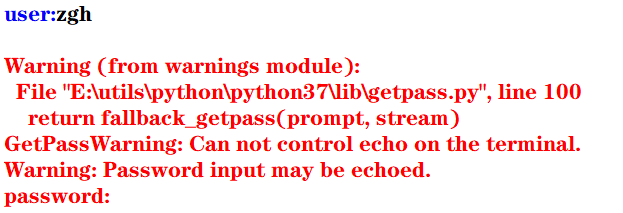但是，在控制台中执行就没问题，看输出结果（可以看到，输入的密码不会显示出来）：
user:zgh

二：重定向标准输出到一个文件的示例
这种重定向由控制台完成，而与Python本身无关。

格式： 程序 > 输出文件

其目的是将显示屏从标准输出中分离，并将输出文件与标准输出关联，即程序的执行结果将写入输出文件，而不是发送到显示屏中显示

首先准备一个test.py文件（代码如下）
import sys,random

n = int(sys.argv)
for i in range(n):
print(random.randrange(0,100))

然后在PowerShell中：python test.py 100 > scores.txt 记住，切记，一定要注意：千万能省略python，这样写./test.py 100 > scores.txt会出现问题，生成的scores文件中会没有任何内容！！！（原因未知）
然后在当前目录下，100个[0,100)范围内的的整数生成在scores.txt文件中了
三：重定向文件到标准输入

格式： 程序 < 输入文件

其目的是将控制台键盘从标准输入中分离，并将输入文件与标准输入关联，即程序从输入文件中读取输入数据，而不是从键盘中读取输入数据

准备一个average.py文件（代码如下）
import sys

total =0.0
count = 0
for line in sys.stdin:
count += 1
total += float(line)

avg = total/count
print("average:",avg)

然后问题总是不期而至， 在PowerShell中：python average.py < scores.txt，会报错，PowerShell会提示你：“<”运算符是为将来使用而保留的。 很无奈，我只能使用cmd了，然后得出结果
四：管道

格式： 程序1 | 程序2 | 程序3 | … | 程序4

其目的是将程序1的标准输出连接到程序2的标准输入， 将程序2的标准输出连接到程序3的标准输入，以此类推

例如： 打开cmd，输入python test.py 100 | average.py，其执行结果等同于上面两个例子中的命令
使用管道更加简洁，且不用创建中间文件，从而消除了输入流和输出流可以处理的数据大小的限制，执行效率更高
五：过滤器
使用操作系统实用程序more逐屏显示数据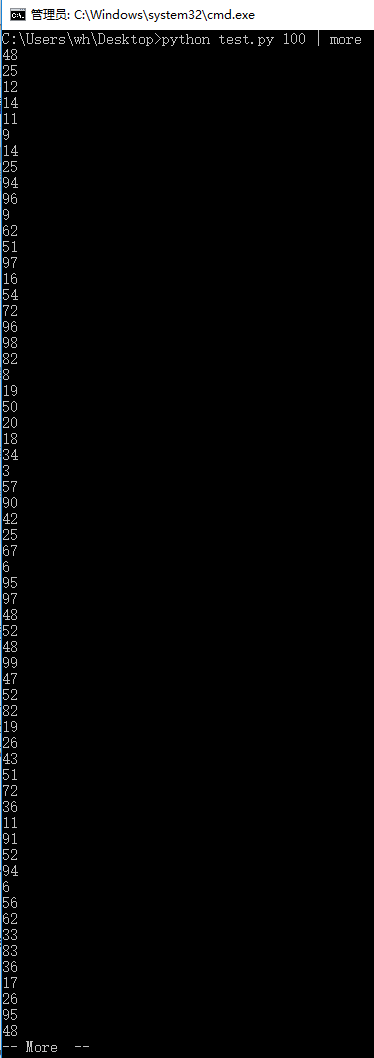使用操作系统实用程序sort排序输出数据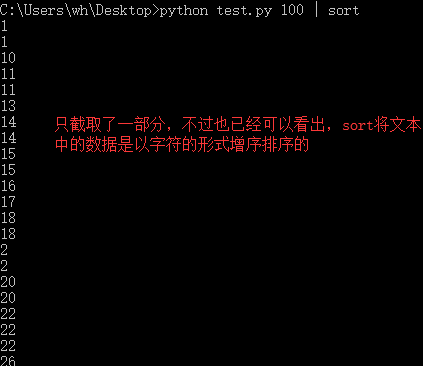more和sort都可以在一个语句中使用
填空题：1、2

print(value, ..., sep = ' ', end = '\n', file = sys.stdout, flush = False)
sep（分隔符，默认为空格）end（换行符，即输入的末尾是个啥）file（写入到指定文件流，默认为控制台sys.stdout）flush（指定是否强制写入到流）

1
>>> print(1,2,3,4,5,sep='-',end='!')
1-2-3-4-5!

2
>>> for i in range(10):
print(i,end=' ')

0 1 2 3 4 5 6 7 8 9

例题及上机实践：2~5
2. 尝试修改例6.2编写的命令行参数解析的程序，解析命令行参数所输入边长的值，计算并输出正方形的周长和面积

argparse模块用于解析命名的命令行参数，生成帮助信息的Python标准模块

例6.2：解析命令行参数所输入的长和宽的值，计算并输出长方形的面积
import argparse

parser = argparse.ArgumentParser()
parser.add_argument('--length', default = 10, type = int, help = '长度')
parser.add_argument('--width', default = 5, type = int, help = '宽度')

args = parser.parse_args()
area = args.length * args.width
print('面积 = ', area)

input()#加这一句是为了可以看到输出结果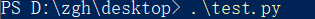输出：面积 = 50
如果在执行这个模块时，加入两个命令行参数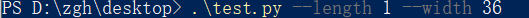输出：面积 = 36

基本上看了上面这个例子后，就可以理解argparse的用法了

本题代码：
import argparse

parser = argparse.ArgumentParser()
parser.add_argument('--length', default = 10, type = int, help = '长度')

args = parser.parse_args()
area = args.length ** 2
perimeter = 4 * args.length
print('面积 = {0}，周长 = {1}'.format(area,perimeter))

input()#加这一句是为了可以看到输出结果


在PowerShell中输入.\test.py 不给命令行参数，输出是以默认值来计算的 输出：面积 = 100，周长 = 40
给命令行参数：.\test.py --length 1 输出：面积 = 1，周长 = 4
3. 尝试修改例6.8编写读取并输出文本文件的程序，由命令行第一个参数确认所需输出的文本文件名

f = open(file, mode = 'r' , buffering = -1, encoding = None)
file是要打开或创建的文件名，如果文件不在当前路径，需指出具体路径mode是打开文件的模式，模式有： ‘r’（只读） ‘w’（写入，写入前删除就内容） ‘x’（创建新文件，如果文件存在，则导致FileExistsError） ‘a’（追加） ‘b’（二进制文件） ‘t’（文本文件，默认值） ‘+’（更新，读写）buffering表示是否使用缓存（缓存为-1，表示使用系统默认的缓冲区大小）encoding是文件的编码

例6.8：读取并输出文本文件
import sys

filename = sys.argv#就读取本文件，骚的呀皮
f = open(filename, 'r', encoding = 'utf-8')

line_no = 0
while True:
line_no += 1
if line:
print(line_no, ":", line)
else:
break
f.close()

输出（代码输出的就是本python文件）：
1 : import sys

2 :

3 : filename = sys.argv#就读取本文件，骚的呀皮

4 : f = open(filename, 'r', encoding = 'utf-8')

5 :

6 : line_no = 0

7 : while True:

8 :     line_no += 1

10 :     if line:

11 :         print(line_no, ":", line)

12 :     else:

13 :         break

14 : f.close()

15 :


本题代码：
对例题代码进行些许修改就可以了，首先将上例中的第二个语句改为：filename = sys.argv，再考虑下面怎么进行
准备一个用来测试的文件test.txt：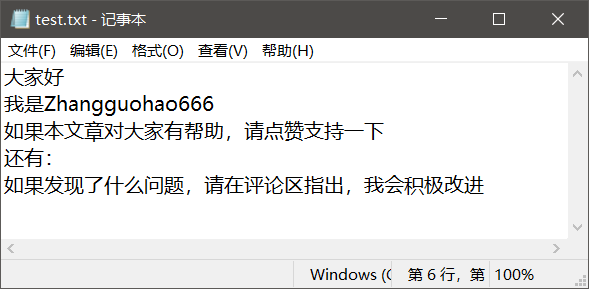对于这个文件要注意一点（你们很可能回出现这个问题！！！），win10默认创建的文本文件的字符编码是ANSI
代码怎么写，有两种：
将test.txt文本文件的编码修改为utf-8，代码如上所说 记事本方式打开test.txt文件，点击文件，点击另存为，看到下方的编码（修改为utf-8）test.txt就用默认的ANSI编码方式，再将上例代码的第三个语句修改为f = open(filename, 'r', encoding = 'ANSI')
在PowerShell中输入：./test.py test.txt 输出：
1 : ﻿大家好

2 : 我是Zhangguohao666

3 : 如果本文章对大家有帮助，请点赞支持一下

4 : 还有：

5 : 如果发现了什么问题，请在评论区指出，我会积极改进

4. 尝试修改例6.9编写利用with语句读取并输出文本文件的程序，由命令行第一个参数确认所需输出的文本文件名

为了简化操作，Python语言中与资源相关的对象可以实现上下文管理协议，可以使用with语句，确保释放资源。 with open(file,mode) as f:

例6.9：利用with语句读取并输出文本文件
import sys

filename = sys.argv

line_no = 0
with open(filename, 'r', encoding = 'utf-8') as f:
for line in f:
line_no += 1
print(line_no, ":", line)
f.close()


基本上，看这个例子，就可以上手with语句了

本题代码：
还是上一题准备的文本文件， 代码一（文本文件的编码为默认的ANSI）：
import sys

filename = sys.argv

line_no = 0
with open(filename, 'r', encoding = 'ANSI') as f:
for line in f:
line_no += 1
print(line_no, ":", line)
f.close()


代码二（将文本文件的编码修改为utf-8）：
import sys

filename = sys.argv

line_no = 0
with open(filename, 'r', encoding = 'utf-8') as f:
for line in f:
line_no += 1
print(line_no, ":", line)
f.close()


本题的输出，我再不要脸的放一次吧：
1 : ﻿大家好

2 : 我是Zhangguohao666

3 : 如果本文章对大家有帮助，请点赞支持一下

4 : 还有：

5 : 如果发现了什么问题，请在评论区指出，我会积极改进

5. 尝试修改例6.12编写标准输出流重定向的程序，从命令行第一个参数中获取n的值，然后将0-n，0-n的2倍值，2的0-n次幂的列表打印输出到out.log文件中
例6.12：从命令行第一个参数中获取n的值，然后将0-n，2的0-n次幂的列表打印输出到out.log文件中

标准输入流文件对象：sys.stdin， 默认值为sys.__stdin__标准输出流文件对象：sys.stdout， 默认值为sys.__stdout__错误输出流文件对象（标准错误流文件对象）：sys.stderr 默认值为sys.__stderr__

书中给的代码是这样的：
import sys

n = int(sys.argv)
power = 1
i = 0

f = open('out.log', 'w')
sys.stdout = f

while i <= n:
print(str(i), ' ', str(power))
power = 2*power
i += 1
sys.stdout = sys.__stdout__

如果使用的编辑器是PyCharm（现在大多数编辑器会帮你对代码进行优化和处理一些隐患），运行书中的这个代码没有问题。
但是： 若使用的编辑器是python自带的IDLE，运行这个代码有问题！
第一：out.log文件会生成，但是没有东西 （发现文件关闭不了（就是×不掉）， 确定是文件没关闭（f.close()）的原因）
第二：控制台没有输出’done’语句（估计是IDLE编辑器处理不了__stdout__这个值）
经过研究后，发现（基于IDLE编辑器）： 如果在上面的代码中加入f.close()后，该输入的东西都成功输入进out.log文件了， 但是， 还有一个问题 控制台依旧没有输出’done’语句 经过一步步的断点调试（就是手动写print） 发现sys.stdout = sys.__stdout__不会执行
然后进行改动后，就可以了，代码如下： （既然__stdout__不好使，就使用中间变量）
import sys

n = int(sys.argv)
power = 1
i = 0

output = sys.stdout
f = open('out.log', 'w')
sys.stdout = f

while i <= n:
print(str(i), ' ', str(power))
power = 2*power
i += 1

f.close()
sys.stdout = output
print('done!')#这一句是用来检测上面的代码是否成功执行



问题虽然解决，但是原因没有彻底弄清楚，求助。。。。。。

本题代码：
import sys

n = int(sys.argv)
power = 1
i = 0

output = sys.stdout
f = open('out.log', 'w')
sys.stdout = f

while i <= n:
print(str(i), ' ',  str(2*i),  ' ', str(power))
power = 2*power
i += 1

f.close()
sys.stdout = output
print('done!')#这一句是用来检测上面的代码是否成功执行


比如时输入的命令行参数是6 输出：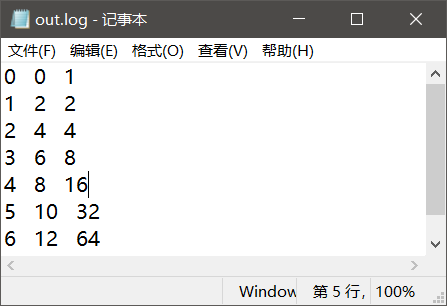案例研究：21点扑克牌游戏
https://blog.csdn.net/Zhangguohao666/article/details/103948545
通过21点扑克牌游戏的设计和实现，了解使用Python数据类型、控制流程和输入输出
第七章 错误和异常处理

Python语言采用结构化的异常处理机制捕获和处理异常

而我感觉，Python在这方面的知识点其实和Java的差不多
几个例题
一：程序的错误和异常处理
语法错误

指源代码中的拼写错误，这些错误导致Python编译器无法把Python源代码转换为字节码，故也称之为编译错误

运行时错误

在解释执行过程中产生的错误

例如：
程序中没有导入相关的模块，NameError程序中包括零除运算，ZeroDivisionError程序中试图打开不存在的文件，FileNotFoundError
逻辑错误

程序可以执行（程序运行本身不报错），但执行结果不正确。 对于逻辑错误，Python解释器无能为力，需要用户根据结果来调试判断

大部分由程序错误而产生的错误和异常一般由Python虚拟机自动抛出。另外，在程序中如果判断某种错误情况，可以创建相应的异常类的对象，并通过raise语句抛出
>>> a = -1
>>> if(a < 0): raise ValueError("数值不能为负数")

Traceback (most recent call last):
File "<pyshell#9>", line 1, in <module>
if(a < 0): raise ValueError("数值不能为负数")
ValueError: 数值不能为负数
>>>


在程序中的某个方法抛出异常后，Python虚拟机通过调用堆栈查找相应的异常捕获程序。如果找到匹配的异常捕获程序（即调用堆栈中的某函数使用try…except语句捕获处理），则执行相应的处理程序（try…except语句中匹配的except语句块）

如果堆栈中没有匹配的异常捕获程序，则Python虚拟机捕获处理异常，在控制台打印出异常的错误信息和调用堆栈，并中止程序的执行

二：try …except…else…finally
try:
可能产生异常的语句
except Exception1:
发生Exception1时执行的语句
except (Exception2,Exception3):
发生Exception2或Exception3时执行的语句
except Exception4 as e:
发生Exception4时执行的语句，Exception4的实例是e
except:
捕获其他所有异常
else:
无异常时执行的语句
finally:
不管异常发生与否都保证执行的语句

except语句可以写多个，但是要注意一点：系统是自上而下匹配发生的异常，所以用户需要将带有最具体的（即派生类程度最高的）异常类的except写在前面
三：创建自定义异常，处理应用程序中出现的负数参数的异常

自定义异常类一般继承于Exception或其子类。自定义异常类的名称一般以Error或Exception为后缀

>>> class NumberError(Exception):
def __init__(self,data):
Exception.__init__
(self,data)
self.data = data
def __str__(self):
return self.data + '：非法数值（<0）'

>>>
>>> def total(data):
total = 0
for i in data:
if i < 0: raise NumberError(str(i))
total += 1

>>>
>>> data1 = (44, 78, 90, 80, 55)
>>> print("sum: ",total(data1))
sum:  5
>>>
>>> data2 = (44, 78, 90, 80, -1)
>>> print("sum: ",total(data2))
Traceback (most recent call last):
File "<pyshell#24>", line 1, in <module>
print("sum: ",total(data2))
File "<pyshell#18>", line 4, in total
if i < 0: raise NumberError(str(i))
NumberError: -1：非法数值（<0）
>>>

四：断言处理
用户在编写程序时，在调试阶段往往需要判断代码执行过程中变量的值等信息：
用户可以使用print()函数打印输出结果也可以通过断点跟踪调试查看变量但使用断言更加灵活
assert语句和AssertionError
断言的声明：
assert <布尔表达式> 即：if __debug__: if not testexpression: raise AssertionErrorassert <布尔表达式>,<字符串表达式> 即：if __debug__: if not testexpression: raise AssertionError(data) 字符串表达式（即data）是断言失败时输出的失败消息
__debug__也是布尔值，Python解释器有两种：调试模式和优化模式
调试模式：__debug__ == True优化模式：__debug__ == False
在学习中，对于执行一个py模块（比如test.py）我们通常在cmd中这么输入python test.py，而这默认是调试模式。 如果我们要使用优化模式来禁用断言来提高程序效率，我们可以加一个运行选项-O，在控制台中这么输入python -O test.py
看一下断言的示例吧，理解一下用法：
a =int(input("a: "))
b =int(input("b: "))
assert b != 0, '除数不能为零'
c = a/b
print("a/b = ", c)

cmd出场： 输入正确数值时：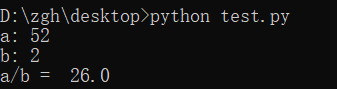输入错误数值时：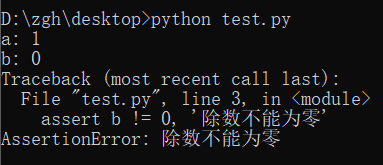禁用断言，并且输入错误数值时：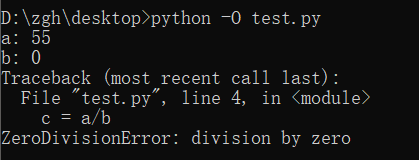案例研究：使用调试器调试Python程序
https://blog.csdn.net/Zhangguohao666/article/details/103948568
了解使用Python调试器调试程序的方法
第八章 函数和函数式编程

一些知识点总结和几个例题
Python中函数的分类：
内置函数 在程序中可以直接使用标准库函数 Python语言安装程序同时会安装若干标准库，例如math、random等第三方库函数 Python社区提供了许多其它高质量的库，在下载、安装这些库后，通过import语句可以导入库用户自定义函数
函数名为有效的标识符（命名规则为全小写字母，可以使用下划线增加可阅读性，例如my_func()）函数可以使用return返回值 如果函数体中包含return语句，则返回值， 否则不返回，即返回值为空（None），无返回值的函数相当于其它编程语言中的过程
调用函数之前程序必须先执行def语句，创建函数对象
内置函数对象会自动创建import导入模块时会执行模块中的def语句，创建模块中定义的函数Python程序结构顺序通常为import语句>函数定义>全局代码
一：产生副作用的函数，纯函数
打印等腰三角形
n = int(input("行数："))

def print_star(n):
print((" * " * n).center(50))

for i in range(1, 2*n, 2):
print_star(i)

输出：
行数：5
*
*  *  *
*  *  *  *  *
*  *  *  *  *  *  *
*  *  *  *  *  *  *  *  *

上面代码中的print_star()是一个产生副作用的函数，其副作用是向标准输出写入若干星号

副作用：例如读取键盘输入，产生输出，改变系统的状态等在一般情况下，产生副作用的函数相当于其它程序设计语言中的过程，可以省略return语句

定义计算并返回第n阶调和数（1+1/2+1/3+…+1/n）的函数，输出前n个调和数
def harmonic(n):
total = 0.0
for i in range(1, n+1):
total += 1.0/i

n = int(input("n："))

print("输出前n个调和数的值：")
for i in range(1, n+1):
print(harmonic(i))

输出：
 n：8
输出前n个调和数的值：
1.0
1.5
1.8333333333333333
2.083333333333333
2.283333333333333
2.4499999999999997
2.5928571428571425
2.7178571428571425

上面代码中的harmonic()是纯函数

纯函数：给定同样的实际参数，其返回值唯一，且不会产生其它的可观察到的副作用

注意：编写同时产生副作用和返回值的函数通常被认为是不良编程风格，但有一个例外，即读取函数。例如，input()函数既可以返回一个值，又可以产生副作用（从标准输入中读取并消耗一个字符串）
二：传递不可变对象、可变对象的引用
实际参数值默认按位置顺序依次传递给形式参数。如果参数个数不对，将会产生错误
在调用函数时：
若传递的是不可变对象（例如：int、float、bool、str对象）的引用，则如果函数体中修改对象的值，其结果实际上是创建了一个新的对象
i = 1

def func(i,n):
i += n
return i

print(i)#1
func(i,10)
print(i)#1

执行函数func()后，i依旧为1，而不是11
若传递的是可变对象（例如：list对象）的引用，则在函数体中可以直接修改对象的值
import random

def shuffle(a):
n = len(a)
for i in range(n):
r = random.randrange(i,n)
a[i],a[r] = a[r],a[i]

a = [1,2,3,4,5]
print("初始：",a)
shuffle(a)
print("调用函数后：",a)

输出：
初始： [1, 2, 3, 4, 5]
调用函数后： [1, 5, 4, 3, 2]

三：可选参数，命名参数，可变参数，强制命名参数
可选参数
在声明函数时，如果希望函数的一些参数是可选的，可以在声明函数时为这些参数指定默认值
>>> def babbles(words, times=1):
print(words * times)

>>> babbles('Hello')
Hello
>>>
>>> babbles("Hello", 2)
HelloHello
>>>

注意到一点：必须先声明没有默认值的形参，然后再声明有默认值的形参，否则报错。 这是因为在函数调用时默认是按位置传递实际参数的。
怎么理解上面那句话呢？
默认是按位置传递实际参数（如果有默认值的形参在左边，无默认值的形参在右，那么在调用函数时，你的实参该怎么传递呢？）
命名参数
位置参数：当函数调用时，实参默认按位置顺序传递形参命名参数（关键字参数）：按名称指定传入的参数 参数按名称意义明确 传递的参数与顺序无关 如果有多个可选参数，则可以选择指定某个参数值
基于期中成绩和期末成绩，按照指定的权重计算总评成绩
>>> def my_sum(mid_score, end_score, mid_rate = 0.4):
score = mid_score*mid_rate + end_score*(1-mid_rate)
print(format(score,'.2f'))

>>> my_sum(80,90)
86.00
>>> my_sum(mid_score = 80,end_score = 90)
86.00
>>> my_sum(end_score = 90,mid_score = 80)
86.00
>>>

可变参数
在声明函数时，可以通过带星号的参数（例如：def func(* param)）向函数传递可变数量的实参，调用函数时，从那一点后所有的参数被收集为一个元组在声明函数时，可以通过带双星号的参数（例如：def func(** param)）向函数传递可变数量的实参，调用函数时，从那一点后所有的参数被收集为一个字典
利用带星的参数计算各数字的累加和
>>> def my_sum(a,b,*c):
total = a+b
for i in c:
total += i

>>> print(my_sum(1,2))
3
>>> print(my_sum(1,2,3,4,5,6))
21

利用带星和带双星的参数计算各数字的累加和
>>> def my_sum(a,b,*c,**d):
total = a+b
for i in c:
total += i
for key in d:
total += d[key]

>>> print(my_sum(1,2))
3
>>> print(my_sum(1,2,3,4))
10
>>> print(my_sum(1,2,3,4,male=1,female=2))
13

强制命名参数
在带星号的参数后面声明参数会导致强制命名参数（Keyword-only），然后在调用时必须显式使用命名参数传递值因为按位置传递的参数默认收集为一个元组，传递给前面带星号的可变参数
>>> def my_sum(*, mid_score, end_score, mid_rate = 0.4):
score = mid_score*mid_rate + end_score*(1-mid_rate)
print(format(score,'.2f'))

>>> my_sum(mid_score=80,end_score=90)
86.00
>>> my_sum(end_score=90,mid_score=80)
86.00
>>> my_sum(80,90)
Traceback (most recent call last):
File "<pyshell#47>", line 1, in <module>
my_sum(80,90)
TypeError: my_sum() takes 0 positional arguments but 2 were given
>>>

四：全局语句global示例，非局部语句nonlocal示例，输出局部变量和全局变量
在函数体中可以引用全局变量，但是要为定义在函数外的全局变量赋值，需要使用global语句
pi = 2.1415926
e = 2.7182818

def my_func():
global pi
pi = 3.14
print("global pi = ", pi)
e = 2.718
print("local e = ", e)

print('module pi = ', pi)
print('module e = ', e)
my_func()
print('module pi = ', pi)
print('module e = ', e)

输出：
module pi =  2.1415926
module e =  2.7182818
global pi =  3.14
local e =  2.718
module pi =  3.14
module e =  2.7182818

在函数体中可以定义嵌套函数，在嵌套函数中如果要为定义在上级函数体的局部变量赋值，可以使用nonlocal
def outer_func():
tax_rate = 0.17
print('outer function tax rate is ',tax_rate)
def inner_func():
nonlocal tax_rate
tax_rate = 0.01
print('inner function tax rate is ',tax_rate)
inner_func()
print('outer function tax rate is ',tax_rate)

outer_func()

输出：
outer function tax rate is  0.17
inner function tax rate is  0.01
outer function tax rate is  0.01

输出局部变量和全局变量
内置函数locals()，局部变量列表内置函数globals()，全局变量列表
五：获取和设置最大递归数
在sys模块中，函数getrecursionlimit()和setrecursionlimit()用于获取和设置最大递归次数
>>> import sys
>>> sys.getrecursionlimit()
1000
>>> sys.setrecursionlimit(666)
>>> sys.getrecursionlimit()
666
>>>

六：三个有趣的内置函数：eval()、exec()、compile()
eval
对动态表达式进行求值，返回值eval(expression, globals=None, locals=None) expression是动态表达式的字符串 globals和locals是求值时使用的上下文环境的全局变量和局部变量，如果不指定，则使用当前运行上下文
>>> x = 2
>>> str_func = input("请输入表达式：")
请输入表达式：x**2+2*x+1
>>> eval(str_func)
9
>>>

exec
可以执行动态表达式，不返回值exec(str, globals=None, locals=None)
>>> exec("for i in range(10): print(i, end=' ')")
0 1 2 3 4 5 6 7 8 9
>>>

compile
编译代码为代码对象，可以提高效率compile(source, filename, mode) source为代码语句的字符串；如果是多行语句，则每一行的结尾必须有换行符\n filename为包含代码的文件 mode为编码方式，可以为'exec'（用于语句序列的执行），可以为'eval'（用于表达式求值），可以为'single'（用于单个交互语句）
>>> co = compile("for i in range(10): print(i, end=' ')", '', 'exec')
>>> exec(co)
0 1 2 3 4 5 6 7 8 9
>>>

七：map()，filter()
map(f, iterable,…)，将函数f应用于可迭代对象，返回结果为可迭代对象
示例1：
>>> def is_odd(x):
return x%2 == 1

>>> list(map(is_odd,range(5)))
[False, True, False, True, False]
>>>

示例2：
>>> list(map(abs,[1,-2,3,-4,5,-6]))
[1, 2, 3, 4, 5, 6]
>>>

示例3：
>>> list(map(str,[1,2,3,4,5]))
['1', '2', '3', '4', '5']
>>

示例4：
>>> def greater(x,y):
return x>y

>>> list(map(greater,[1,5,7,3,9],[2,8,4,6,0]))
[False, False, True, False, True]
>>>

filter(f, iterable)，将函数f应用于每个元素，然后根据返回值是True还是False决定保留还是丢弃该元素，返回结果为可迭代对象
示例1（返回个位数的奇数）：
>>> def is_odd(x):
return x%2 == 1

>>> list(filter(is_odd, range(10)))
[1, 3, 5, 7, 9]
>>>

示例2（返回三位数的回文）：
>>> list(filter(is_palindrome, range(100, 1000)))
[101, 111, 121, 131, 141, 151, 161, 171, 181, 191, 202, 212, 222, 232, 242, 252, 262, 272, 282, 292, 303, 313, 323, 333, 343, 353, 363, 373, 383, 393, 404, 414, 424, 434, 444, 454, 464, 474, 484, 494, 505, 515, 525, 535, 545, 555, 565, 575, 585, 595, 606, 616, 626, 636, 646, 656, 666, 676, 686, 696, 707, 717, 727, 737, 747, 757, 767, 777, 787, 797, 808, 818, 828, 838, 848, 858, 868, 878, 888, 898, 909, 919, 929, 939, 949, 959, 969, 979, 989, 999]
>>>

八：Lambda表达式和匿名函数

匿名函数广泛应用于需要函数对象作为参数、函数比较简单并且只使用一次的场合

格式：
lambda arg1,arg2... : <expression>

其中，arg1、arg2等为函数的参数，<expression>为函数的语句，其结果为函数的返回值
示例1（计算两数之和）：
>>> f = lambda x,y : x+y
>>> type(f)
<class 'function'>
>>> f(1,1)
2
>>>

示例2（返回奇数）：
>>> list(filter(lambda x:x%2==1, range(10)))
[1, 3, 5, 7, 9]
>>>

示例3（返回非空元素）：
>>> list(filter(lambda s:s and s.strip(), ['A', '', 'B', None, 'C', ' ']))
['A', 'B', 'C']
>>>

补充：
strip()用来去除头尾字符、空白符（\n,\r,\t,’’,即换行、回车、制表、空格）lstrip()用来去除开头字符、空白符rstrip()用来去除结尾字符、空白符
再补充一点：
\n到下一行的开头\r回到这一行的开头
示例4（返回大于0的元素）：
>>> list(filter(lambda x:x>0, [1,0,-2,8,5]))
[1, 8, 5]
>>>

示例5（返回元素的平方）：
>>> list(map(lambda x:x*x, range(10)))
[0, 1, 4, 9, 16, 25, 36, 49, 64, 81]
>>>

九：operator模块和操作符函数

Python内置操作符的函数接口，它定义了对应算术和比较等操作的函数，用于map()、filter()等需要传递函数对象作为参数的场合，可以直接使用而不需要使用函数定义或者Lambda表达式，使得代码更加简洁

示例1（concat(x,y)对应于x+y）：
>>> import operator
>>> a = 'hello'
>>>> operator.concat(a, ' world')
'hello world'

实例2（operator.gt对应于操作符>）：
>>> import operator
>>> list(map(operator.gt, [1,5,7,3,9],[2,8,4,6,0]))
[False, False, True, False, True]
>>>

十：functools.reduce()，偏函数functools.partial()，sorted()
functools.reduce()

functools.reduce(func, iterable[, iterable[, initializer]])
使用指定的带两个参数的函数func对一个数据集合的所有数据进行下列操作：使用第一个和第二个数据作为参数用func()函数运算，得到的结果再与第三个数据作为参数用func()函数运算，依此类推，最后得到一个结果可选的initialzer为初始值

示例：
>>> import functools,operator
15
25
5050
>>>
>>> functools.reduce(operator.mul, range(1,11))
3628800

偏函数functools.partial()

functools.partial(func, *arg, **keywords)
通过把一个函数的部分参数设置为默认值的方式返回一个新的可调用（callable）的partial对象主要用于设置预先已知的参数，从而减少调用时传递参数的个数

示例（2的n次方）：
>>> import functools,math
>>> pow2 = functools.partial(math.pow, 2)
>>> list(map(pow2, range(11)))
[1.0, 2.0, 4.0, 8.0, 16.0, 32.0, 64.0, 128.0, 256.0, 512.0, 1024.0]
>>>

十一：sorted()

sorted(iterable, *, key=None, reverse=False)
iterable是待排序的可迭代对象key是比较函数（默认为None，按自然顺序排序）reverse用于指定是否逆序排序

示例1（数值。默认自然排序）：
>>> sorted([1,6,4,-2,9])
[-2, 1, 4, 6, 9]
>>> sorted([1,6,4,-2,9], reverse=True)
[9, 6, 4, 1, -2]
>>> sorted([1,6,4,-2,9], key=abs)
[1, -2, 4, 6, 9]

示例2（字符串，默认按字符串字典序排序）：
>>> sorted(['Dod', 'cat', 'Rabbit'])
['Dod', 'Rabbit', 'cat']
>>> sorted(['Dod', 'cat', 'Rabbit'], key=str.lower)
['cat', 'Dod', 'Rabbit']
>>> sorted(['Dod', 'cat', 'Rabbit'], key=len)
['Dod', 'cat', 'Rabbit']

示例3（元组，默认按元组的第一个元素排序）：
>>> sorted([('Bob', 75), ('Adam', 92), ('Lisa', 88)])
[('Adam', 92), ('Bob', 75), ('Lisa', 88)]
>>> sorted([('Bob', 75), ('Adam', 92), ('Lisa', 88)], key=lambda t:t)
[('Bob', 75), ('Lisa', 88), ('Adam', 92)]

十二：函数装饰器
这玩意就很有意思了，很Java语言中的注解是很相像的
示例1：
import time,functools

def timeit(func):
def wrapper(*s):
start = time.perf_counter()
func(*s)
end = time.perf_counter()
print('运行时间：', end - start)
return wrapper

@timeit
def my_sum(n):
sum = 0
for i in range(n): sum += i
print(sum)

if __name__ == '__main__':
my_sum(10_0000)

结果：
4999950000
运行时间： 0.013929100000000028

怎么理解上面的代码呢？
首先，timeit()返回的是wrapper，而不是执行（没有小括号）@timeit相当于，在调用my_sum()的前一刻，会执行这么个语句：my_sum = timeit(my_sum)
示例2：
def makebold(fn):
def wrapper(*s):
return "<b>" + fn(*s) + "</b>"
return wrapper

def makeitalic(fn):
def wrapper(*s):
return "<i>" + fn(*s) + "</i>"
return wrapper

@makebold
@makeitalic
def htmltags(str1):
return str1

print(htmltags('Hello'))


输出：
<b><i>Hello</i></b>

选择题：1~5
1
>>> print(type(lambda:None))
<class 'function'>

2
>>> f = lambda x,y:x*y
>>> f(12, 34)
408

3
>>> f1 = lambda x:x*2
>>> f2 = lambda x:x**2
>>> print(f1(f2(2)))
8

4
>>> def f1(p, **p2):
print(type(p2))

>>> f1(1, a=2)
<class 'dict'>

5
>>> def f1(a,b,c):
print(a+b)

>>> nums = (1,2,3)
>>> f1(*nums)
3

思考题：4~11
4
>>> d = lambda p:p*2
>>> t = lambda p:p*3
>>> x = 2
>>> x = d(x)
>>> x = t(x)
>>> x = d(x)
>>> print(x)
24

5
>>> i = map(lambda x:x**2, (1,2,3))
>>> for t in i:
print(t, end=' ')

1 4 9

6
>>> def f1():
"simple function"
pass

>>> print(f1.__doc__)
simple function

7
>>> counter = 1
>>> num = 0
>>> def TestVariable():
global counter
for i in (1, 2, 3) : counter += 1
num = 10

>>> TestVariable()
>>> print(counter, num)
4 0

8
>>> def f(a,b):
if b==0 : print(a)
else : f(b, a%b)

>>> print(f(9,6))
3
None

求最大公约数
9
>>> def aFunction():
"The quick brown fox"
return 1

>>> print(aFunction.__doc__[4:9])
quick

10
>>> def judge(param1, *param2):
print(type(param2))
print(param2)

>>> judge(1, 2, 3, 4, 5)
<class 'tuple'>
(2, 3, 4, 5)

11
>>> def judge(param1, **param2):
print(type(param2))
print(param2)

>>> judge(1, a=2, b=3, c=4, d=5)
<class 'dict'>
{'a': 2, 'b': 3, 'c': 4, 'd': 5}

上机实践：2~5
2. 编写程序，定义一个求阶乘的函数fact(n)，并编写测试代码，要求输入整数n（n>=0）。请分别使用递归和非递归方式实现
递归方式：
def fact(n):
if n == 0 :
return 1
return n*fact(n-1)

n = int(input("请输入整数n（n>=0）："))
print(str(n)+" ! =  " + str(fact(n)))


非递归方式：
def fact(n):
t = 1
for i in range(1,n+1):
t *= i
return t

n = int(input("请输入整数n（n>=0）："))
print(str(n)+" ! =  " + str(fact(n)))


输出：
请输入整数n（n>=0）：5
5 ! =  120

3. 编写程序，定义一个求Fibonacci数列的函数fib(n)，并编写测试代码，输出前20项（每项宽度5个字符位置，右对齐），每行输出10个。请分别使用递归和非递归方式实现
递归方式：
def fib(n):
if (n == 1 or n == 2):
return 1
return fib(n-1)+fib(n-2)

for i in range(1,21):
print(str(fib(i)).rjust(5,' '),end = ' ')
if i %10 == 0:
print()

非递归方式：
def fib(n):
if (n == 1 or n == 2):
return 1
n1 = n2 = 1
for i  in range(3,n+1):
n3 = n1+n2
n1 = n2
n2 = n3
return n3

for i in range(1,21):
print(str(fib(i)).rjust(5,' '),end = ' ')
if i %10 == 0:
print()

输出：
    1     1     2     3     5     8    13    21    34    55
89   144   233   377   610   987  1597  2584  4181  6765

4. 编写程序，利用可变参数定义一个求任意个数数值的最小值的函数min_n(a,b,*c)，并编写测试代码。例如对于“print(min_n(8, 2))”以及“print(min_n(16, 1, 7, 4, 15))”的测试代码
def min_n(a,b,*c):
min_number = a if(a < b) else b
for n in c:
if n < min_number:
min_number = n
return min_number

print(min_n(8, 2))
print(min_n(16, 1, 7, 4, 15))

输出：
2
1

5. 编写程序，利用元组作为函数的返回值，求序列类型中的最大值、最小值和元素个数，并编写测试代码，假设测试代码数据分别为s1=[9, 7, 8, 3, 2, 1, 55, 6]、s2=[“apple”, “pear”, “melon”, “kiwi”]和s3="TheQuickBrownFox"
def func(n):
return (max(n),min(n),len(n))

s1=[9, 7, 8, 3, 2, 1, 55, 6]
s2=["apple", "pear", "melon", "kiwi"]
s3="TheQuickBrownFox"

for i in (s1,s2,s3):
print("list = ", i)
t = func(i)
print("最大值 = {0}，最小值 = {1}，元素个数 = {2}".format(t, t, t))

输出：
list =  [9, 7, 8, 3, 2, 1, 55, 6]
最大值 = 55，最小值 = 1，元素个数 = 8
list =  ['apple', 'pear', 'melon', 'kiwi']
最大值 = pear，最小值 = apple，元素个数 = 4
list =  TheQuickBrownFox
最大值 = x，最小值 = B，元素个数 = 16

案例研究：井字棋游戏
https://blog.csdn.net/Zhangguohao666/article/details/103280740
了解Python函数的定义和使用

由于本文的内容太多了，导致了两个很不好的结果， 一是：在网页中打开本篇博客的加载时间太长了，明显的卡顿很影响阅读体验； 二是：本人在对本篇文章进行更新或者修改内容时，卡的要死。 遂， 将本文第八章后面的很多内容拆分到新的文章中，望大家理解

第九章 面向对象的程序设计

《Python程序设计与算法基础教程（第二版）》江红 余青松，第九章课后习题答案
第十章 模块和客户端

《Python程序设计与算法基础教程（第二版）》江红 余青松，第十章课后习题答案
第十一章 算法与数据结构基础

《Python程序设计与算法基础教程（第二版）》江红 余青松，第十一章课后习题答案
第十二章 图形用户界面

我对图形用户界面基本无兴趣，无特殊情况，基本不打算碰这方面内容
案例研究：简易图形用户界面计算器
Python实现简易图形用户界面计算器
第十三章 图形绘制

与上一章相同，我对于图形绘制的兴趣也基本没有，尝试做了2-7题，就完全没兴趣做下去了
图形绘制模块：tkinter
2. 参考例13.2利用Canvas组件创建绘制矩形的程序，尝试改变矩形边框颜色以及填充颜色
from tkinter import *

root = Tk()
c = Canvas(root, bg = 'white', width = 130, height = 70)
c.pack()

c.create_rectangle(10, 10, 60, 60, fill = 'red')
c.create_rectangle(70, 10, 120, 60, fill = 'green', outline = 'blue', width = 5)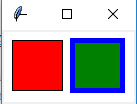创建画布对象：
root = Tk() 创建一个Tk根窗口组件rootc = Canvas(root, bg = 'white', width = 130, height = 70) 创建大小为200 * 100、背景颜色为白色的画布c.pack() 调用组件pack()方法，调整其显示位置和大小
绘制矩形：
c.create_rectangle(x0, y0, x1, y1, option, ...)

（x0，y0）是左上角的坐标（x1，y1）是右下角的坐标c.create_rectangle(70, 10, 120, 60, fill = 'green', outline = 'blue', width = 5) 用蓝色边框、绿色填充矩形，边框宽度为5
3. 参考例13.3利用Canvas组件创建绘制椭圆的程序，尝试修改椭圆边框样式、边框颜色以及填充颜色
from tkinter import *

root = Tk()
c = Canvas(root, bg = 'white', width = 280, height = 70)
c.pack()

c.create_oval(10, 10, 60, 60, fill = 'green')
c.create_oval(70, 10, 120, 60, fill = 'green', outline = 'red', width = 5)
c.create_oval(130, 25, 180, 45, dash = (10,))
c.create_oval(190, 10, 270, 50, dash = (1,), width = 2)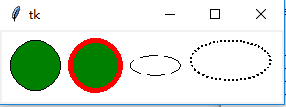绘制椭圆
c.create_oval(x0, y0, x1, y1, option, ...)

（x0，y0）是左上角的坐标（x1，y1）是右下角的坐标c.create_oval(70, 10, 120, 60, fill = 'green', outline = 'red', width = 5) 绿色填充、红色边框，宽度为5c.create_oval(130, 25, 180, 45, dash = (10,)) 虚线椭圆
4. 参考例13.4利用Canvas组件创建绘制圆弧的程序，尝试修改圆弧样式、边框颜色以及填充颜色
from tkinter import *

root = Tk()
c = Canvas(root, bg = 'white', width = 250, height = 70)
c.pack()

c.create_arc(10, 10, 60, 60, style = ARC)
c.create_arc(70, 10, 120, 60, style = CHORD)
c.create_arc(130, 10, 180, 60, style = PIESLICE)
for i in range(0, 360, 60):
c.create_arc(190, 10, 240, 60, fill = 'green', outline = 'red', start = i, extent = 30)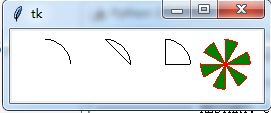绘制圆弧：
c.create_arc(x0, y0, x1, y1, option, ...)

（x0，y0）是左上角的坐标（x1，y1）是右下角的坐标选项start（开始角度，默认为0）和extend（圆弧角度，从start开始逆时针旋转，默认为90度）决定圆弧的角度范围选项start用于设置圆弧的样式
5. 参考例13.5利用Canvas组件创建绘制线条的程序，尝试修改线条样式和颜色
from tkinter import *

root = Tk()
c = Canvas(root, bg = 'white', width = 250, height = 70)
c.pack()

c.create_line(10, 10, 60, 60, arrow = BOTH, arrowshape = (3, 4, 5))
c.create_line(70, 10, 95, 10, 120, 60, fill = 'red')
c.create_line(130, 10, 180, 10, 130, 60, 180, 60, fill = 'green', width = 10, arrow = BOTH, joinstyle = MITER)
c.create_line(190, 10, 240, 10, 190, 60, 240, 60, width = 10)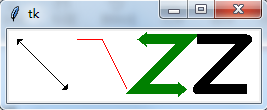绘制线条：
c.create_line(x0, y0, x1, y1, ..., xn, yn, option, ...)

（x0，y0）,（x1，y1），…，（xn，yn）是线条上各个点的坐标
6. 参考例13.6利用Canvas组件创建绘制多边形的程序，尝试修改多边形的形状、线条样式和填充颜色
from tkinter import *

root = Tk()
c = Canvas(root, bg = 'white', width = 250, height = 70)
c.pack()

c.create_polygon(35, 10, 10, 60, 60, 60, fill = 'red', outline = 'green')
c.create_polygon(70, 10, 120, 10, 120, 60, fill = 'white', outline = 'blue')
c.create_polygon(130, 10, 180, 10, 180, 60, 130, 60, outline = 'blue')
c.create_polygon(190, 10, 240, 10, 190, 60, 240, 60, fill = 'white', outline = 'black')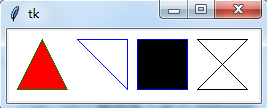绘制多边形：
c.create_polygon(x0, y0, x1, y1, ..., option, ...)

（x0，y0）,（x1，y1），…，（xn，yn）是多边形上各个顶点的坐标
7. 参考例13.7利用Canvas组件创建绘制字符串和图形的程序，绘制y = cos(x) 的图形
绘制字符串：
c.create_text(x, y, option, ...)

（x，y）是字符串放置的中心位置
y = sin(x)
from tkinter import *
import math

WIDTH, HEIGHT = 510, 210
ORIGIN_X, ORIGIN_Y = 2, HEIGHT/2 #原点

SCALE_X, SCALE_Y = 40, 100 #x轴、y轴缩放倍数
ox, oy = 0, 0
x, y = 0, 0
arc = 0 #弧度
END_ARC = 360 * 2 #函数图形画两个周期

root = Tk()
c = Canvas(root, bg = 'white', width = WIDTH, height = HEIGHT)
c.pack()

c.create_text(200, 20, text = 'y = sin(x)')
c.create_line(0, ORIGIN_Y, WIDTH, ORIGIN_Y)
c.create_line(ORIGIN_X, 0, ORIGIN_X, HEIGHT) #绘制x轴，y轴
for i in range(0, END_ARC+1, 10):
arc = math.pi * i / 180
x = ORIGIN_X + arc * SCALE_X
y = ORIGIN_Y - math.sin(arc) * SCALE_Y
c.create_line(ox, oy, x, y)
ox, oy = x, y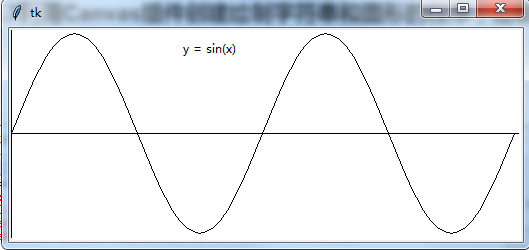y = cos(x)
from tkinter import *
import math

WIDTH, HEIGHT = 510, 210
ORIGIN_X, ORIGIN_Y = 2, HEIGHT/2 #原点

SCALE_X, SCALE_Y = 40, 100 #x轴、y轴缩放倍数
ox, oy = 0, 0
x, y = 0, 0
arc = 0 #弧度
END_ARC = 360 * 2 #函数图形画两个周期

root = Tk()
c = Canvas(root, bg = 'white', width = WIDTH, height = HEIGHT)
c.pack()

c.create_text(200, 20, text = 'y = cos(x)')
c.create_line(0, ORIGIN_Y, WIDTH, ORIGIN_Y)
c.create_line(ORIGIN_X, 0, ORIGIN_X, HEIGHT)
for i in range(0, END_ARC+1, 10):
arc = math.pi * i / 180
x = ORIGIN_X + arc * SCALE_X
y = ORIGIN_Y - math.cos(arc) * SCALE_Y
c.create_line(ox, oy, x, y)
ox, oy = x, y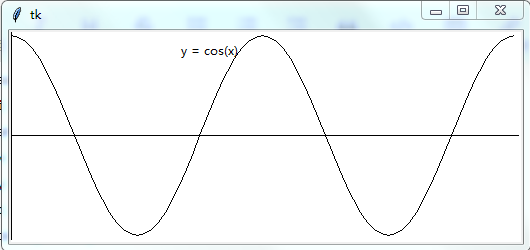图形绘制模块：turtle
…

后面章节内容：未完待续…
第十四章 数值日期和时间处理

第十五章 字符串和文本处理

第十六章 文件和数据交换

第十七章 数据访问

第十八章 网络编程和通信

第十九章 并行计算：进程、线程和协程

第二十章 系统管理
展开全文• 为将要创建的画板（即窗口）设置大小，figsize=(宽度,高度)，dpi=像素点大小 plt.figure(1) #创建第1块画板 ax1 = plt.subplot(211) #将第1块画板划分为2行1列组成的区块，并获取到第1块区域 plt.xxx() #在该区域...
1 plt.scatter() 描绘点
plt.scatter(x, y,s=, c=20, '#ff0000', marker='o', cmap=, norm=,
vmin=, vmax=, alpha=, linewidths=, verts=, edgecolors=,data=)

x,y需要绘制的点c点的颜色，可以直接填单色，也可以是一个数组，数组映射于cmap中的颜色。若c=’’，则为透明s点的大小，可以是一个数字，也可以是一个数组，即每个点有对应大小marker点的形状，一共有这些选项(‘o’, ‘v’, ‘^’, ‘<’, ‘>’, ‘8’, ‘s’, ‘p’, ‘*’, ‘h’, ‘H’, ‘D’, ‘d’, ‘P’, ‘X’)cmap需要映射的颜色edgecolors绘制点的边缘色。一般在c=’'时，设置边缘的可绘制空心圆
2 plt.bar() 绘制柱状图
3 plt.contour() 绘制等高线
4 plt.xlim(a, b) 设置x轴的显示范围a~b
5 plt.grid() 绘制网格参考线
6 plt.xlabel(u’x轴’, fontsize=13)
7 plt.title(u’标题’, fontsize=13)
8 plt.set_facecolor(‘none’) 设置背景透明
9 matplotlib 画动态图
1） 方法一
fig = plt.figure()
plt.ion()

try:
ax.lines.remove(line)
except Exception:
pass

lines = ax.plot(x_date, y_date, 'r-', lw=5) #可以在这之前更新x_data和y_data的数据
plt.pause(0.1) #绘图暂停0.1s
plt.pause(0) #防止图片自动关闭

2） 方法二
from matplotlib.animation import FuncAnimation

fig = plt.figure()
xdata, ydata = [],[]
ln, = ax.plot([], [], animated=False) #注意返回的ln后面有的','，ln是在FuncAnimation中的一条线索

def init():
ax.set_xlim() 等初始化
return ln

def update(n):
# 可以根据传入的n来更新xdate，ydate
ln.set_data(xdata, ydata)
return ln

ani = FuncAnimation(fig, update, frames=np.linspace(0, 2*np.pi, 10), init_func=init, blit=True)
plt.show()

# 其中：
FuncAnimation(fig, func, frames, init_func, interval, blit)
# fig:绘制动图画布的名称
# func:自定义的运动函数，也是更新函数
# frames:动画长度，整个动画中所有的帧数，在函数运行的时候，其值会传递给上面的函数
# init_func:初始化函数，自定义起始帧，也就是最开的画面，
# interval:更新频率，单位ms
# bit:为True则只更新改变了的值，为False更新所有值

10 matplotlib 画多个图
plt.figure(figsize=(5,5), dpi=40)  #可选。为将要创建的画板（即窗口）设置大小，figsize=(宽度,高度)，dpi=像素点大小
plt.figure(1) #创建第1块画板
ax1 = plt.subplot(211) #将第1块画板划分为2行1列组成的区块，并获取到第1块区域
plt.xxx() #在该区域画图
# 如果ax1的创建采用fig = plt.figure(1)
ax2 = plt.subplot(212) #将第1块画板划分为2行1列组成的区块，并获取到第2块区域
plt.xxx() #在该区域画图
plt.figure(figsize=(5,5), dpi=40) #可选
plt.figure(2) #创建第2块画板
plt.xxx() #在该区域画图
plt.figure(1) #切换到第1块画板
ax1.xxx() #继续操作对应的区域
plt.show()

11 plt.pcolormesh() 绘制分类，用颜色区分X、Y：是需要绘制的点，可以用 np.mgrid[1:3:3j, 4:6:3j] 生成；
Z：是对应点的标签，标签中值的范围映射于cmap属性中颜色变化的范围；
cmap：是显示的颜色，可以为渐变色，也可以为几种单色。
cm有两种获取方式：
1） 方法一
cm = plt.cm.get_cmap('rainbow')

这里的rainbow是渐变色，颜色的选取可以参考官网
2） 方法二
import matplotlib as mpl
cm = mpl.colors.ListedColormap(['#A0FFA0', '#FFA0A0', '#A0A0FF']) #这里是三种单色
cm = mpl.colors.ListedColormap(['g', 'r', 'b']) #

12 plt.ginput() 通过鼠标在画布上点击，生成数据
参数：
例：
data = plt.ginput(-1)
print(data)
plt.show()
# plt.show() 会抢先执行，也就是会显示出画布之后，再用plt.ginput收集数据，收集完后，会执行它后面的语句。

13 推荐的绘图背景色和对应描点的颜色
点‘#ff0000’, ‘#e100de’, ‘#00e11a’,‘#e1de00’, ‘#00d9e1’, ‘#0060ff’背景‘#5a0000’, ‘#60005f’, ‘#005a0a’,‘#575600’, ‘#005457’, ‘#002460’
对应的颜色如图：14 热度/灰度图像显示
num = mnist.train.images #num为 ‘长x宽’的二维数组
plt.imshow(num) #热成像，num中每一个元素的数字对应着某种颜色
#plt.imshow(num, vmin=0, vmax=1, cmap='gray') 设置颜色映射的上下限，cmap设成gray就是灰度显示，否则默认热度显示
plt.show()
`
展开全文• 滑动窗口就是能够根据指定的单位长度来框住时间序列，从而计算框内的统计指标。相当于一个长度指定的滑块正在刻度尺上面滑动，每滑动一个单位即可反馈滑块内的数据。 看个例子 import numpy as np import pandas as ...
• 前言 想写数据动态可视化很久了，但是网上竟然没有一份能直接用的代码，...动图的核心函数是matplotlib.animation.FuncAnimation，基本用法： anim = animation.funcanimation(fig, animate, init_func=init, ...Python
• var.set("预测的结果是：" + answer) if __name__ == "__main__": creat_windows() stock_predict.py import numpy as np import matplotlib.pyplot as plt import tensorflow as tf import pandas as pd import ...LSTM模型 股票预测 python实现
• ## Python 数据科学入门教程：Matplotlib

万次阅读 多人点赞 2017-01-13 11:45:55
Matplotlib 入门教程 来源：Introduction to Matplotlib and basic line 译者：飞龙 协议：CC BY-NC-SA 4.0 在线阅读 PDF格式 EPUB格式 MOBI格式 代码仓库 第一章 Matplotlib 简介 欢迎阅读 Python...python
• Python中matplotlib实时画图 　https://blog.csdn.net/zhanghao3389/article/details/82685072 Python中的plt.rcParams[]使用 　http://www.cnblogs.com/pacino12134/p/9776882.html 　pyplot使用rc配置文件来自定义...python 动态图表
• matplotlib概述 matplotlib是python的一个绘图库。使用它可以很方便的绘制出版质量级别的图形。...图形对象(图形窗口) 子图 刻度定位器 刻度网格线 半对数坐标 散点图 填充 条形图 饼图 等高线图 热成像图 ...python 可视化
• 对于较为简单的时间序列预测问题，可以使用Exponential Smoothing和ARIMA等传统模型非常方便地求解。然而，对于复杂的时间序列预测问题，LSTM不失为一种很好的选择。因此，本文旨在探讨如何利用LSTM神经网络求解时间...Keras LSTM
• 使用指南 ...matplotlib可以画布上可视化你的数据，而画布可以是Windows窗口、Jupyter窗口等等,每个画布包含有一个或多个坐标轴，这里所指的坐标轴可以是X-Y的直角坐标轴，也可以是角度-半径的极坐标..可视化 python
• ## Python可视化库matplotlib(超详细)

千次阅读 多人点赞 2021-06-30 21:21:42
超详细Matplotlib笔记Matplotlib 简介开发环境搭建为什么要学习Matplotlib绘制基础图形绘制流程认识Matplotlib图像结构实现基础绘图功能设置标签文字和线条粗细解决中文乱码&符号不正常显示绘制直线绘制折线折线...python
• ## Python--matplotlib绘图

万次阅读 多人点赞 2018-01-04 21:29:22
折线图在我的工作中用到的比较多，因为写论文时，经常要在不同的参数环境下比较算法的运行时间。同时，折线图也是最简单的，所以我拿它“开刀”，来介绍pyplot模块最基本的操作。 现在绘制一个简单的二维折线图，...
• 最近因为毕业设计的原因接触到pyqt5的库，用了一段时间，就想把使用过程中发生的事情记录下来，以供日后学习使用。 0.参考书目 1.《PyQt5快速开发与实战》：pyqt5的内容几乎都是参考了这里。脚本之x下的盗版，这书...pyplot PYQT5
• 一、matplotlib如何嵌入PyQt5中？ 通过matplotlib.backends.backend_qt5agg类连接PyQt5。在实际代码中，我们需要在引用部分加入内容： import matplotlib matplotlib.use("Qt5Agg") # 声明使用QT5 from ...
• 0. 图形窗口 plt . figure ( '' , # 窗口标题栏 figsize = ( 80 , 20 ) , # 窗口大小 dpi = 80 , # 像素密度 facecolor = '' # 图表背景颜色 ) plt . show ( ) 当前窗口相关...
• matplotlib是基于Python的绘图库，广泛用于Python科学计算界。它完整支持二维绘图以及部分支持三维绘图。该绘图库致力于能适应广泛的用户需求。它可以根据所选的用户接口工具来嵌入绘图算法。与此同时，对于使用GTK+...
• ## Matplotlib库简介

千次阅读 2020-08-25 09:46:39
一、Matplotlib绘制图形 我们已经学习过了 NumPy 的统计分析，数据的各项指标都可以以真实的数据提供出来， 有的同学可能有疑问？ 我们为什么要进行可视化？而可视化在数据分析中又占什么样的地位？我们之前大致说过...数据分析 python 可视化
• 恩，翻译至：Matplotlib教程 看看这是个啥？ 简介 Matplotlib是一个类似GNUplot的绘图库。 GNUplot的主要优点是Matplotlib是一个Python模块。 由于python越来越受欢迎，matplotlib也越来越受欢迎。（相辅相成） ...python
• 时间窗口上计算各种统计函数 窗口函数： 滚动统计 obj.rolling().func window 窗口大小 center 窗口是否居中统计 import pandas as pd import numpy as np ser_obj = pd.Series(np.random.randn(1000), ...Python
• matplotlib支持绘制三维点阵、三维曲面、三维线框图： ax3d . scatter ( . . ) # 绘制三维点阵 ax3d . plot_surface ( . . ) # 绘制三维曲面 ax3d . plot_wireframe ( . . ) # 绘制三维线框图 3d散点...数据分析
• Python数据可视化——使用Matplotlib创建散点图 2017-12-27 作者：淡水化合物 转载请注明网址：https://www.cnblogs.com/pengsky2016/p/8126623.html Matplotlib简述： 　Matplotlib是一个用于创建出高质量图表...
• 大家好，最近在研究在搞Python的大作业，有个需求就是利用Matplotlib画几个像模像样的统计图然后合并在一张图中，因为此前很少用这方面的东西，所以折腾了不少时间，今天介绍一下。 subplot多合一 其实，利用python...python 数据分析...